# KR20110123769A - Image identifier matching device - Google Patents

## Info

Publication number
KR20110123769A
KR20110123769A KR1020117021098A KR20117021098A KR20110123769A KR 20110123769 A KR20110123769 A KR 20110123769A KR 1020117021098 A KR1020117021098 A KR 1020117021098A KR 20117021098 A KR20117021098 A KR 20117021098A KR 20110123769 A KR20110123769 A KR 20110123769A
Authority
KR
South Korea
Prior art keywords
quantization
value
image
dimension
values
Prior art date
Application number
KR1020117021098A
Other languages
Korean (ko)
Other versions
KR101398272B1 (en
Inventor
료마 오아미
고타 이와모토
Original Assignee
닛본 덴끼 가부시끼가이샤
Priority date (The priority date is an assumption and is not a legal conclusion. Google has not performed a legal analysis and makes no representation as to the accuracy of the date listed.)
Filing date
Publication date
Priority to JP2009061021 priority Critical
Priority to JPJP-P-2009-061021 priority
Priority to JP2009097863 priority
Priority to JPJP-P-2009-097863 priority
Application filed by 닛본 덴끼 가부시끼가이샤 filed Critical 닛본 덴끼 가부시끼가이샤
Priority to PCT/JP2010/001768 priority patent/WO2010103848A1/en
Publication of KR20110123769A publication Critical patent/KR20110123769A/en
Application granted granted Critical
Publication of KR101398272B1 publication Critical patent/KR101398272B1/en

• 238000004364 calculation methods Methods 0.000 claims description 83
• 230000000875 corresponding Effects 0.000 claims description 23
• 238000009940 knitting Methods 0.000 claims description 8
• 240000001439 Opuntia Species 0.000 claims 2
• 238000000605 extraction Methods 0.000 description 249
• 238000000034 methods Methods 0.000 description 36
• 239000000203 mixtures Substances 0.000 description 19
• 230000000694 effects Effects 0.000 description 17
• 238000010586 diagrams Methods 0.000 description 16
• 238000009826 distribution Methods 0.000 description 13
• 230000001174 ascending Effects 0.000 description 7
• 239000000284 extracts Substances 0.000 description 6
• 241000983672 Ficus natalensis Species 0.000 description 5
• 230000004048 modification Effects 0.000 description 5
• 238000006011 modification reactions Methods 0.000 description 5
• 238000005242 forging Methods 0.000 description 4
• 239000012141 concentrates Substances 0.000 description 3
• 210000003733 Optic Disk Anatomy 0.000 description 2
• 238000006243 chemical reactions Methods 0.000 description 2
• 239000002131 composite materials Substances 0.000 description 2
• 230000002093 peripheral Effects 0.000 description 2
• 230000001360 synchronised Effects 0.000 description 2
• 238000009827 uniform distribution Methods 0.000 description 2
• 230000003044 adaptive Effects 0.000 description 1
• 230000004075 alteration Effects 0.000 description 1
• 239000011651 chromium Substances 0.000 description 1
• 238000007906 compression Methods 0.000 description 1
• 238000003708 edge detection Methods 0.000 description 1
• 238000001914 filtration Methods 0.000 description 1
• 238000009499 grossing Methods 0.000 description 1
• 239000010955 niobium Substances 0.000 description 1
• 238000010606 normalization Methods 0.000 description 1
• 239000002304 perfumes Substances 0.000 description 1
• 239000004065 semiconductors Substances 0.000 description 1
• 241000894007 species Species 0.000 description 1
• 238000001228 spectrum Methods 0.000 description 1
• 239000004544 spot-on Substances 0.000 description 1
• 230000001629 suppression Effects 0.000 description 1

## Images

•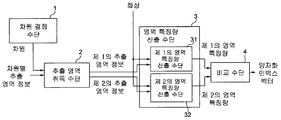•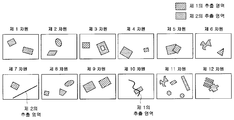•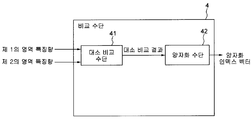•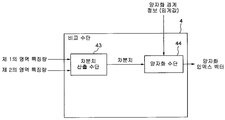•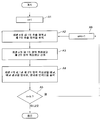•••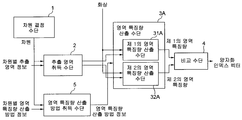•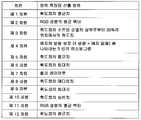•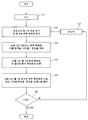••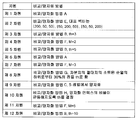•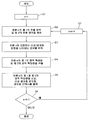•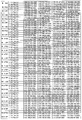••••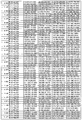••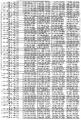•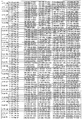••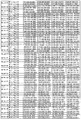••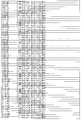•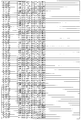•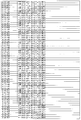•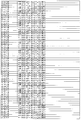•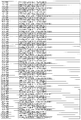•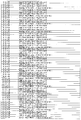•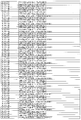•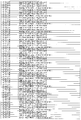•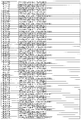•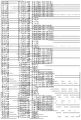•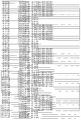••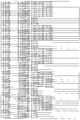•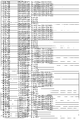••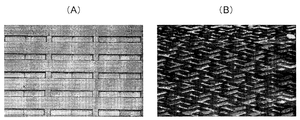•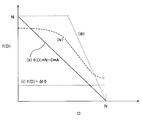••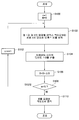•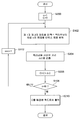••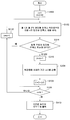•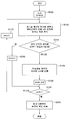•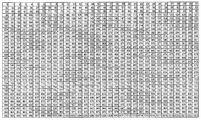•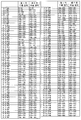•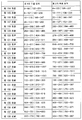••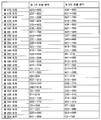•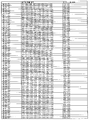•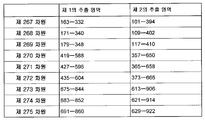•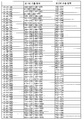•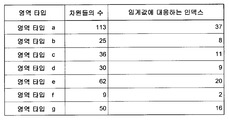•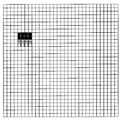•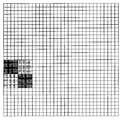•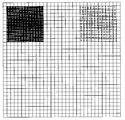•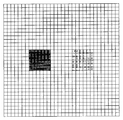•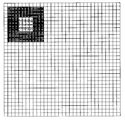••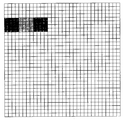## Classifications

• GPHYSICS
• G06COMPUTING; CALCULATING; COUNTING
• G06KRECOGNITION OF DATA; PRESENTATION OF DATA; RECORD CARRIERS; HANDLING RECORD CARRIERS
• G06K9/00Methods or arrangements for reading or recognising printed or written characters or for recognising patterns, e.g. fingerprints
• G06K9/80Combination of image preprocessing and recognition functions
• GPHYSICS
• G06COMPUTING; CALCULATING; COUNTING
• G06FELECTRIC DIGITAL DATA PROCESSING
• G06F16/00Information retrieval; Database structures therefor; File system structures therefor
• G06F16/50Information retrieval; Database structures therefor; File system structures therefor of still image data
• G06F16/58Retrieval characterised by using metadata, e.g. metadata not derived from the content or metadata generated manually
• G06F16/583Retrieval characterised by using metadata, e.g. metadata not derived from the content or metadata generated manually using metadata automatically derived from the content
• GPHYSICS
• G06COMPUTING; CALCULATING; COUNTING
• G06KRECOGNITION OF DATA; PRESENTATION OF DATA; RECORD CARRIERS; HANDLING RECORD CARRIERS
• G06K9/00Methods or arrangements for reading or recognising printed or written characters or for recognising patterns, e.g. fingerprints
• G06K9/00624Recognising scenes, i.e. recognition of a whole field of perception; recognising scene-specific objects
• G06K9/00711Recognising video content, e.g. extracting audiovisual features from movies, extracting representative key-frames, discriminating news vs. sport content
• G06K9/00744Extracting features from the video content, e.g. video "fingerprints", or characteristics, e.g. by automatic extraction of representative shots or key frames
• GPHYSICS
• G06COMPUTING; CALCULATING; COUNTING
• G06KRECOGNITION OF DATA; PRESENTATION OF DATA; RECORD CARRIERS; HANDLING RECORD CARRIERS
• G06K9/00Methods or arrangements for reading or recognising printed or written characters or for recognising patterns, e.g. fingerprints
• G06K9/36Image preprocessing, i.e. processing the image information without deciding about the identity of the image
• G06K9/46Extraction of features or characteristics of the image
• G06K9/52Extraction of features or characteristics of the image by deriving mathematical or geometrical properties from the whole image
• GPHYSICS
• G06COMPUTING; CALCULATING; COUNTING
• G06KRECOGNITION OF DATA; PRESENTATION OF DATA; RECORD CARRIERS; HANDLING RECORD CARRIERS
• G06K9/00Methods or arrangements for reading or recognising printed or written characters or for recognising patterns, e.g. fingerprints
• G06K9/62Methods or arrangements for recognition using electronic means
• G06K9/6201Matching; Proximity measures
• G06K9/6215Proximity measures, i.e. similarity or distance measures

## Abstract

The image identifier used for the combination is generated by the following generation method. First, a region feature amount is extracted from each partial region of a plurality of partial region pairs in the image, and the difference value of the region feature amounts of two partial regions forming a pair is quantized for each partial region pair. In this quantization, if the absolute value of the difference value is smaller than a predetermined prescribed value, the quantization is performed with a specific quantization value. A set having quantized values calculated for each partial region pair as elements is defined as an image identifier used for identification of the image. The image identifier combining device combines the image identifier of the first image and the image identifier of the second image, generated by the generation method described above, so that the weight of the element which is the specific quantization value is reduced.

## Description

Image identifier combination device {IMAGE IDENTIFIER MATCHING DEVICE}

The present invention relates to an apparatus for combining images using an image identifier which is a feature amount (for determining identity) for identifying the image.

An image identifier is an image feature amount (for determining identity) for identifying an image. By comparing the image identifier extracted from one image and the image identifier extracted from another image, it is possible to calculate an identity measure (typically referred to as similarity or distance) in which two images show the same degree from the comparison result. In addition, it is possible to determine whether two images are the same by comparing the calculated identity measure with a threshold value. Here, "the two images are the same" is not limited to the case where the two images are the same at the level of the image signal (pixel value of the pixels constituting the image), but the conversion of the image compression format and the size / word of the image Various modifications such as conversion of the spectra, adjustment of the color tone of the image, various filter processing (sharpening, smoothing, etc.) on the image, local processing of the image (caption superposition, cutout, etc.), recapturing of the image, and the like. This also includes the case where one image is a duplicated image of the other image. By using an image identifier, for example, since a duplicate of a picture or a moving picture that is a collection of pictures can be detected, the picture identifier can be applied to an illegal copy detection system or the like of a picture or a moving picture.

An example of an image identifier is described in patent document 1. As shown in FIG. FIG. 18 is a diagram illustrating a method for extracting an image identifier described in Patent Literature 1. FIG. This picture identifier is a feature vector of a plurality of dimensions (16 dimensions in FIG. 18). Average luminance values are respectively calculated from 32 rectangular areas 244 (of which 16 rectangular areas are drawn in FIG. 18) at predetermined positions in the image 240, and between paired rectangular areas (pairs in FIG. 18). The difference between the average luminance values is calculated by the rectangular region formed by dotted lines 248), and a difference vector 250 of 16 dimensions is obtained. A composite vector is generated for the difference vector 250 by vector transform, and the 16-dimensional quantization index vector obtained by quantizing each dimension of the composite vector is used as an image identifier.

Prior art literature

Patent Literature

Patent Document 1: Japanese Patent Publication No. Hei 8-500471

 The image identifier composed of feature vectors of a plurality of dimensions has a smaller (or less redundancy) information amount of the feature vector as the correlation between the dimensions becomes smaller, so that an identification capability that is enough to identify different images is obtained. Increases. On the contrary, when the correlation between the dimension of the feature vector is large, the amount of information the feature vector has is small (large redundancy), so that the identifying ability is low. Here, the correlation between dimensions is the degree of similarity of occurrence of the feature quantities of the dimension, and mathematically, for example, the correlation coefficient between the probability variables and the mutual information amount when the occurrence of the feature quantities of each dimension is used as the random variable. It is a value calculated as. For this reason, it is preferable that the image identifier composed of the feature vectors of a plurality of dimensions be designed so that the correlation between the dimensions is small.

Image signals (pixel values of pixels constituting an image) have a correlation between local regions of an image. In general, the closer the distance between local regions is, the greater the correlation. In particular, for example, an image in which a certain image pattern appears repeatedly (especially when the image pattern appears repeatedly at regular intervals) (for example, an image of a window of a building arranged in a grid pattern, etc., see FIG. 19A) Or the image (refer to FIG. 19B) which consists of a specific texture, etc., the correlation between local areas of an image becomes large.

[First Problem]

An image identifier composed of a feature vector composed of feature quantities extracted from a plurality of local regions of an image, as described in Patent Literature 1, has a feature amount in each dimension for an image having a large correlation between local regions of the image. Since the shape of the local area | region which extracts this is the same (the rectangular area of the same shape in the example of patent document 1), the correlation between the dimension of the feature amount to be extracted becomes large. For this reason, there is a first problem that the identification capability of the picture identifier (feature vector) is lowered. Note that the same shape here means the same, including the size and angle of the area (tilt or orientation).

For example, about the image (refer FIG. 19A) in which a specific image pattern repeatedly appears, the image comprised by the specific specific texture (refer FIG. 19B), etc., it is described by patent document 1 The image identifier has low identification capability.

[Second Problem]

The second problem of the image identifier described in Patent Document 1 is that since the shape (including size and angle) of the area of each dimension for calculating the feature amount (feature vector) is the same rectangle, the length of the side of the rectangle There is a blind spot on the frequency such that a frequency component having a period equal to or one of its integers cannot be detected. The reason for this is that if an average is taken within a region of a signal component of this particular frequency, it becomes 0 regardless of the magnitude of the signal component, and the signal of the frequency component cannot be detected at all. More specifically, assuming that a frequency having a period equal to the length of a rectangular side is f 0 , the component of frequency nf 0 ( n = 1, 2,3, ...) cannot be detected. For this reason, with respect to the direct current component and the image in which the signal is concentrated on this frequency component, the average value of the pixel values becomes the same as the direct current component, and there is no difference in values between the regions. As a result, the value of all the feature amounts extracted as the difference between the average pixel values between the regions becomes 0 and cannot be identified (the identification capability is significantly reduced). In reality, it is difficult to detect not only the components of the frequency nf 0 ( n = 1, 2,3, ...) but also the constant frequency region in the vicinity thereof, even if the signal components are not concentrated on the specific frequency. Signal component can not be used, and the identifying ability is lowered. In order to alleviate this problem, it is conceivable to increase the value of the frequency f 0 to reduce the signal power falling into the difficult-to-detect frequency band. However, increasing the value of the frequency f 0 means reducing the size of the region, which leads to a deterioration in the robustness of the characteristic amount (the degree to which the characteristic amount does not change with respect to various modification processing and noise). For example, when the area becomes small, the value of the feature amount changes significantly even for a small position shift, and the robustness of the feature amount is lowered. Thus, when using the same rectangular area, it is very difficult to secure robustness while increasing the discrimination ability.

[Objective of the invention]

An object of the present invention is to provide an image identifier combination device capable of solving the problem described above, that is, a problem in that the combination accuracy is lowered in the combination using an image identifier having a low identification capability, which is enough to identify different images.

 Such an image identifier combining device according to an aspect of the present invention includes combining means for combining an image identifier of a first image and an image identifier of a second image. The image identifiers of the first image and the image identifiers of the second image extract the region feature amounts from the respective partial regions of the plurality of partial region pairs in the image, and form the two partial regions paired for each partial region pair. Quantizes a difference value of the region feature amount of the quantized value and quantizes the difference value when the absolute value of the difference value is smaller than a predetermined value. It is generated by a generating method that is used as an image identifier to be used. The combining means combines the image identifier of the first image and the image identifier of the second image in such a way that the weight of the element having the specific quantization value becomes smaller.

 Since the present invention is configured as described above, it is possible to combine the first image and the second image with high precision by using an image identifier with a high identification capability that is capable of identifying different images. In particular, this effect is remarkable for an image having a large correlation between local regions of the image.

 According to the present invention, even in an image in which signals are concentrated at a specific frequency, the combination can be performed using an image identifier in which the identification capability does not decrease.

1 is a block diagram of a first embodiment of the present invention.
2 is a diagram illustrating an example of a pair of extraction areas for each dimension in which the extraction information for each dimension is shown.
3 is a block diagram showing an example of a comparison means in the first embodiment of the present invention.
4 is a block diagram showing another example of the comparison means in the first embodiment of the present invention.
Fig. 5 is a flowchart showing the flow of processing in the first embodiment of the present invention.
6 is a block diagram of an essential part of a second embodiment of the present invention.
7 is a flowchart showing the flow of processing in the second embodiment of the present invention.
8 is a block diagram of a third embodiment of the present invention.
9 is a diagram illustrating an example of a method for calculating region feature quantities for each dimension.
Fig. 10 is a flowchart showing the flow of processing in the third embodiment of the present invention.
Fig. 11 is a block diagram showing a fourth embodiment of the present invention.
12 is a table showing an example of the comparison and quantization method for each dimension.
It is a flowchart which shows the flow of a process of 4th Embodiment of this invention.
FIG. 14A is a table showing dimensional extraction area information used in the fifth and sixth embodiments of the present invention. FIG.
FIG. 14B is a table showing dimension-specific extraction region information used in the fifth and sixth embodiments of the present invention. FIG.
FIG. 14C is a table showing dimension-specific extraction region information used in the fifth and sixth embodiments of the present invention. FIG.
FIG. 14D is a table showing dimensional extraction region information used in the fifth and sixth embodiments of the present invention. FIG.
FIG. 14E is a table showing dimension-specific extraction region information used in the fifth and sixth embodiments of the present invention. FIG.
FIG. 14F is a table showing dimension-specific extraction region information used in the fifth and sixth embodiments of the present invention. FIG.
Fig. 14G is a table showing dimension-specific extraction region information used in the fifth and sixth embodiments of the present invention.
Fig. 14H is a table showing dimension-specific extraction region information used in the fifth and sixth embodiments of the present invention.
FIG. 14I is a table showing dimension-specific extraction region information used in the fifth and sixth embodiments of the present invention. FIG.
Fig. 14J is a table showing dimensional extraction area information used in the fifth and sixth embodiments of the present invention.
It is a table | surface which shows the area characteristic amount calculation method information for each dimension used by 5th Embodiment of this invention.
Fig. 15B is a table showing the area characteristic amount calculation method information for each dimension used in the fifth embodiment of the present invention.
It is a table | surface which shows the area characteristic amount calculation method information for each dimension used by 5th Embodiment of this invention.
It is a table | surface which shows the area characteristic amount calculation method information for each dimension used by 5th Embodiment of this invention.
It is a table | surface which shows the area characteristic amount calculation method information for each dimension used by 5th Embodiment of this invention.
It is a table | surface which shows the area characteristic amount calculation method information for each dimension used by 6th Embodiment of this invention.
Fig. 16B is a table showing the dimension feature amount calculation method information for each dimension used in the sixth embodiment of the present invention.
Fig. 16C is a table showing the area characteristic amount calculation method information for each dimension used in the sixth embodiment of the present invention.
It is a table | surface which shows the area characteristic amount calculation method information for each dimension used by 6th Embodiment of this invention.
It is a table | surface which shows the area characteristic amount calculation method information for each dimension used by 6th Embodiment of this invention.
FIG. 17A is a table showing dimensional comparison and quantization method information used in the fifth and sixth embodiments of the present invention. FIG.
FIG. 17B is a table showing dimensional comparison and quantization method information used in the fifth and sixth embodiments of the present invention. FIG.
FIG. 17C is a table showing dimensional comparison and quantization method information used in the fifth and sixth embodiments of the present invention. FIG.
FIG. 17D is a table showing dimensional comparison and quantization method information used in the fifth and sixth embodiments of the present invention. FIG.
FIG. 17E is a table showing dimensional comparison and quantization method information used in the fifth and sixth embodiments of the present invention. FIG.
It is a figure which shows the extraction method of the image identifier described in patent document 1. FIG.
Fig. 19 is a diagram illustrating an example of an image in which the correlation between local regions becomes large.
20 is a graph showing an example of the forging ratio increasing function f (D).
Fig. 21 is a block diagram of combining means for combining quantization index vectors.
Fig. 22 is a flowchart showing a process example of the combining means for combining the quantization index vectors.
Fig. 23 is a flowchart of another processing example of the combining means for combining the quantization index vectors.
Fig. 24 is a flowchart showing still another example of processing of the combining means for combining the quantization index vectors.
Fig. 25 is a flowchart showing still another processing example of the combining means for combining the quantization index vectors.
Fig. 26 is a flowchart illustrating another processing example of the combining means for combining the quantization index vectors.
Fig. 27 is a diagram showing an example of an index given to 1024 blocks that can divide an image into 32 vertical directions and 32 horizontal directions.
It is a table | surface which shows the area | region which belongs to one type among the area | region corresponding to each dimension of 7th Embodiment of this invention.
FIG. 28B is a table showing a region belonging to one type of regions corresponding to each dimension of the seventh embodiment of the present invention. FIG.
FIG. 28C is a table illustrating a region belonging to one type of regions corresponding to each dimension of the seventh embodiment of the present invention. FIG.
FIG. 28D is a table illustrating a region belonging to one type of regions corresponding to each dimension of the seventh embodiment of the present invention. FIG.
Fig. 28E is a table showing a region belonging to one type of regions corresponding to each dimension of the seventh embodiment of the present invention.
FIG. 28F is a table illustrating a region belonging to one type of regions corresponding to each dimension of the seventh embodiment of the present invention. FIG.
Fig. 28G is a table showing a region belonging to one type of regions corresponding to each dimension of the seventh embodiment of the present invention.
FIG. 29 is a table showing a relationship between an area type of each dimension, the number of dimensions, and an index corresponding to a threshold value.
It is a figure which shows an example of the 1st, 2nd extraction area | region of the dimension of area | region type a.
It is a figure which shows an example of the 1st, 2nd extraction area | region of the dimension of area | region type b.
It is a figure which shows an example of the 1st, 2nd extraction area | region of the dimension of area | region type c.
It is a figure which shows an example of the 1st, 2nd extraction area | region of the dimension of area | region type d.
It is a figure which shows an example of the 1st, 2nd extraction area | region of the dimension of area | region type e.
It is a figure which shows an example of the 1st, 2nd extraction area | region of the dimension of area | region type f.
It is a figure which shows an example of the 1st, 2nd extraction area | region of the dimension of area | region type g.

[First Embodiment]

[Configuration of First Embodiment]

Next, 1st Embodiment of this invention is described in detail with reference to drawings.

Referring to FIG. 1, an image identifier extracting apparatus according to a first embodiment of the present invention includes a feature identifier (more specifically, a quantization index vector) that includes a feature vector having a plurality of dimensions with respect to an input image. As a system that outputs. The image identifier extracting apparatus includes a dimension determining means 1, an extracting region obtaining means 2, an area feature variable calculating means 3, and a comparing means 4.

The dimension determining means 1 next determines the dimension of the feature vector to be extracted and supplies it to the extraction region acquisition means 2. The dimension determination means 1 sequentially supplies the dimension of the feature vector to extract, and the component after the extraction area | region acquisition means 2 extracts the feature quantity corresponding to the supplied dimension. For example, when the feature vector is configured in N dimensions, the dimension determination means 1 may sequentially supply the extraction region acquisition means 2 from the first dimension to the Nth dimension. Finally, once all the dimensions of the feature vector are supplied, they can be supplied in any order of dimensions. Plural dimensions may be supplied in parallel.

The extraction region acquisition means 2 is supplied with dimension-specific extraction region information as an input separately from the dimension supplied from the dimension determination means 1.

The dimension-specific extraction region information is information indicating a predetermined pair of the first extraction region and the second extraction region, which are associated for each dimension of the feature vector. The first and second extraction regions have the following characteristics as essential conditions.

[Required Conditions of First and Second Extraction Areas]

The prerequisites of the first and second extraction regions are that the relative positions of the extraction region pairs differ among the dimensions, and that the combination of the shape of the extraction region pairs among the dimensions differs.

For each of the dimensions indicated by the dimension-specific extraction information, an example of a pair of extraction regions that satisfy the above essential condition is shown in FIG. 2. It differs from the extraction area of the image identifier shown in FIG. 18, and the knitting of the shape of the pair of extraction areas is different among each dimension. Here, different shapes may refer to the same shape at different angles (for example, the second extraction region of the first dimension and the first extraction region of the seventh dimension) of FIG. 2, or similar shapes of different sizes (eg, A second extraction region of the first dimension of FIG. 2 and a second extraction region of the ninth dimension). In addition, it is a minimum condition that at least one dimension in which the pair of extraction areas has a combination of different shapes is included in the entire dimension of the feature vector. The more the dimension of (shape) of pair of extraction area | region differs from each other, the more preferable. This is because the more the dimensions of different shapes (combination) of the pairs of extraction regions are different from each other, the smaller the correlation between the dimensions than those of the feature vector, and the higher the discrimination ability. For example, the shapes of the pairs of extraction regions may differ from one another among all dimensions of the feature vector.

The first extraction region and the second extraction region in the dimension do not have to be the same shape as in the ninth dimension of FIG. 2, and the shapes may be different as in the other dimensions of FIG. 2. When the shape of the first extraction region and the second extraction region in each dimension is different, the correlation between the feature amount extracted from the first extraction region and the second extraction region is small, and the identification capability is increased. This is therefore desirable. Moreover, since the likelihood of becoming blind spots with respect to the same frequency at the same time as the first extraction region and the second extraction region is lowered, the discrimination ability is increased.

The shape of each extraction region is arbitrary. For example, any complex shape, such as the second extraction region of the sixth dimension of FIG. 2, may also be acceptable. If the extraction region consists of a plurality of pixels of the image, line segments or curves may also be allowed, for example, as in the seventh or tenth dimension of FIG. 2. Further, for example, a plurality of small regions in which the extraction regions are not continuous, such as the first extraction region in the eighth dimension, the first and second extraction regions in the eleventh dimension, and the first extraction region in the twelfth dimension It may be composed of regions. As such, when the feature vector includes an extraction region of a complicated shape, the correlation between the dimensions of the feature quantities extracted therefrom can be lowered, so that the identifying ability can be increased.

For example, as in the fifth dimension of FIG. 2, a part of the first extraction region and the second extraction region may overlap each other. In addition, any one of the extraction region pairs may be contained in the other. As such, by allowing redundancy in the pair of extraction regions, more patterns (relative positions, distances) can be taken in the extraction region pairs, so that patterns that can reduce the correlation between dimensions can be increased, thereby improving identification ability. The probability of making it higher is increased.

In addition, different from the extraction area of the image identifier shown in FIG. 18, as in each of the dimensions shown in FIG. 2, the extraction areas may partially overlap each other. As shown in the extraction area of the image identifier shown in Fig. 18, if the extraction area is exclusively taken between the dimensions, the possible patterns of the extraction area pairs are limited. As shown in Fig. 2, by allowing the overlapping of the extraction regions between the dimensions, the pattern that can reduce the correlation between the dimensions can be increased, thereby increasing the possibility of making the identification ability higher. However, if there are too many overlapping portions of the extraction area between the dimensions, the correlation between the dimensions becomes large and the identification ability becomes low. Therefore, this is not desirable.

In addition, in the case of incorporating the extraction areas of all dimensions, an extraction area is taken so that the area where the feature amount is not extracted in the image becomes smaller (that is, almost the full screen of the image can be covered). It is preferable. As shown in Fig. 18, when a large number of regions in which the feature amount is not extracted in the image is included, much information included in the image signal (pixel values of pixels constituting the image) is not used, and the identification capability is not increased. . In the case of incorporating the extraction regions of all dimensions, the extraction region is taken into account by taking the extraction region so that the region where the feature amount is not extracted in the image becomes smaller (that is, almost the full screen of the image can be covered). As more information can be reflected in the feature amount, the identification ability can be enhanced. In addition, when the extraction areas of all dimensions are integrated, it is preferable that the extraction areas are not deflected and uniformly acquired from the entire image. However, if there is a high probability that local processing such as subtitle superimposition is performed on any particular region, it is preferable to obtain the extraction region avoiding the region. In addition, since the peripheral region of the edge of the image often does not include the feature portion of the image, it is preferable to obtain the extraction region avoiding the peripheral region.

In addition, it is preferable that the size, relative position (distance, direction) of the extraction region follow a constant distribution (for example, a uniform distribution), because the relative position (distance, direction) is according to a uniform distribution, This is because the extraction area is not deflected with respect to the distance or direction, so that the extraction area does not concentrate at a specific distance or direction, and thus more diversity can be achieved. In addition, the closer the relative position is, the larger the correlation between the regions is, so that the larger the difference in shape is, the better the relative position is.

The extraction region information for each dimension may be any form of information as long as the first extraction region and the second extraction region for each dimension are uniquely identifiable information. Since the extraction area must always be the same area for any size or aspect ratio image, the dimension-specific extraction area information can be obtained in the same extraction area for any size or aspect ratio image. It should be information of. For example, the dimension-specific extraction region information describes the position and shape of the extraction region with respect to an image having a predetermined size and aspect ratio (for example, an image having a width of 320 pixels × a width of 240 pixels). You may. In this case, for an image input at any arbitrary size and aspect ratio, the image is first resized to have a predetermined size and aspect ratio, and then according to the position and shape of the extraction region described in the extraction region information for each dimension. The extraction region may be specified. Conversely, the extraction region may be specified by converting the position and shape of the extraction region described in the dimensional extraction region information corresponding to the image of the arbitrary size and aspect ratio of the input image.

Information representing each extraction region included in the dimension-specific extraction region information is, for an image of a predetermined size and aspect ratio (for example, an image of 320 pixels wide × 240 pixels wide), It may be information describing a set of coordinate values of all pixels constituting the extraction region. The information indicating each extraction region included in the dimension-specific extraction region information may be information that describes, as parameters, the position and shape of the extraction region with respect to an image of a predetermined size and aspect ratio. For example, if the shape of the extraction area is a rectangle, the information may describe the coordinate values of the four corners of the rectangle. In addition, when the shape of the extraction area is a circle, the information may describe the coordinate value and the radius value of the center of the circle.

In addition, by using the seed of the pseudo random number as the extraction area information for each dimension, starting from the species in the extraction area acquisition means 2 to generate a pseudo random number, extraction of different shapes according to the random number You can also choose to create an area (for example, the four corners of a rectangle are determined by random numbers). Specifically, the dimension-specific extraction region can be obtained, for example, in the following order.

(1) It is supplied as information of extraction area for each paper dimension of pseudo random numbers.

(2) The dimension is set to n = 1.

(3) A pseudo random number is generated to determine four corners of the rectangle of the first extraction region of the dimension n.

(4) A pseudo random number is generated to determine four corners of the rectangle of the second extraction region of the dimension n.

(5) The dimension is set to n = n + 1, and the procedure returns to (3).

Since the extraction region is determined based on the random number, the generated extraction region has a different shape for each dimension. In addition, since the same random number is generated every time (for any image) when the pseudo random number is the same, the same extraction area is reproduced for the different images.

The extraction region acquisition means 2 is information indicating a first extraction region and a second extraction region corresponding to the dimension supplied from the dimension determination means 1 from the dimension-specific extraction region information supplied as an input. Is obtained and supplied to the extraction region representative value calculation means (3).

In addition to the input from the extraction area acquisition means 2 (information indicating the first extraction area and the second extraction area), the area feature variable calculating means 3 serves as an input of the image identifier to be extracted. The image is supplied. The area feature variable calculating means 3 has a first area feature variable calculating means 31 and a second area feature variable calculating means 32. The area feature variable calculating means 3 uses the first area feature variable calculating means 31 to extract the first extraction region supplied from the extraction area acquisition means 2 for each dimension from the image supplied as the input. Based on the information to be shown, the feature amount of the first extraction region is calculated as the first feature feature and supplied to the comparison means 4. Moreover, the area feature variable calculating means 3 extracts the 2nd feature supplied from the extraction area acquisition means 2 for every dimension from the image supplied as an input using the 2nd area feature variable calculating means 32. As shown in FIG. Based on the information indicating the area, the feature amount of the second extraction area is calculated as the second area feature amount and supplied to the comparison means 4.

Further, in order to specify each extraction area for the input image based on the information indicating the first extraction area and the second extraction area, the area feature variable calculating means 3, if necessary, The image is resized to have a predetermined size and aspect ratio of the dimensional extraction area information.

The area feature variable calculating means 3 calculates the area feature variable of each extraction region by using pixel values of the pixel group included in each extraction region. Here, the pixel value is a value of a signal that each pixel of the image has, and is a scalar amount or a vector amount. For example, when the image is a luminance image, the pixel value is a luminance value (scalar amount), and when the image is a color image, the pixel value is a vector amount indicating a color component. For example, when a color image is an RGB image, a pixel value is a three-dimensional vector amount of R component, G component, and B component. When the color image is a YCbCr image, the pixel value is a three-dimensional vector amount of the Y component, the Cb component, and the Cr component.

In order to calculate the area feature amount of the extraction area, the method of calculating the extraction area (the first extraction area and the second extraction area) of the dimension is constant (the same calculation method is used for any input image). ), Any method may be used.

The area feature amount to be calculated may be a scalar amount or a vector amount. For example, when the pixel value is a scalar amount such as a luminance value, the area feature amount may be calculated as an average value, a median value, a maximum number of bins, a maximum value, a minimum value, or the like (all are scalar amounts). For example, the pixel values included in the extraction region may be sorted, and the pixel values at positions having a predetermined ratio from the upper or lower portion of the distribution (sorted permutation) may be calculated as region feature amounts (this is also a scalar amount). ). More specifically, the case where P% of the percentage is a predetermined ratio (for example P = 25%) will be described. The pixel values (luminance values) of N pixels in the extraction area are sorted in ascending order, and the set of pixel values (luminance values) sorted in ascending order is Y (i) = (Y (0), Y ( 1), Y (2),... , Y (N-1)}. Here, the pixel value at the position of P% from the lower part of the permutation sorted in ascending order becomes, for example, Y (floor (N × P / 100)), and this value is obtained as the area feature amount of the extraction region. do. In addition, floor () is a function that performs truncation after the decimal point. Here, the region feature amount calculated by applying this formula (Y (floor (N × P / 100))) to the luminance value of the pixel included in the extraction region is referred to as "percentile luminance value feature amount". .

In addition, when the pixel value is a vector amount such as a color component, first, they may be converted into a scalar amount by any method, and then the area feature amount may be calculated by the method described above. For example, when the pixel value is a three-dimensional vector amount of RGB components, first, the area values may be calculated by the method described above after converting them into luminance values that are scalar amounts. In addition, when the pixel value is a vector amount, it is also possible to use, for example, an average vector of pixel values included in the extraction area as the area feature amount.

For example, you may perform arbitrary calculations (differential calculation, filtering operation), such as edge detection and template matching, with respect to an extraction area | region, and may use the calculation result as area | region feature amount. For example, it may be a two-dimensional vector amount indicating the direction of the edge (direction of the gradient), or a scalar amount indicating similarity with any template.

In addition, a histogram representing the color distribution included in the extraction region, the directional distribution of the edges, and the intensity distribution of the edges may be obtained as the area feature amounts (all are vector quantities).

In addition, various feature quantities defined in ISO / IEC 15938-3 may be used, which is a dominant color, a color layout, a scalable color, a color structure. Edge Histogram, Homogeneous Texture, Texture Browsing, Region Shape, Contour Shape, Shape 3D, Parametric Motion Motion, and Motion Activity.

The comparison means 4 compares the first area feature amount supplied from the area feature variable calculating means 3 with the second area feature amount for each dimension, and quantizes the result of comparison to obtain the quantization index. Outputs Since the comparison means 4 outputs a quantization index for each dimension, a quantization index vector consisting of quantization indices of a plurality of dimensions is finally output.

The comparison means 4 may use any method for comparing and quantizing the first region feature amount with the second region feature amount. The number of quantization indexes for each dimension is also arbitrary.

The comparison means 4, for example, when the area feature amount is a scalar amount (for example, an average value of luminance values), and compares the magnitude and the quantization index is +1 when the first area feature amount is large. In this case, the quantization index may be set to −1, and the comparison result may be quantized to a quantization index of two values of +1 and −1. Here, if the first region feature amount of the dimension n is Vn1 and the second region feature amount is Vn2, the quantization index Qn of the dimension n can be calculated by the following equation.

[Equation 1]

Qn = + 1 (for Vn1> Vn2)

-1 (for Vn1≤Vn2)

Here, FIG. 3 shows a more detailed configuration diagram of the comparison means 4 in the case where the comparison means 4 performs the comparison and quantization based on Equation 1 above.

Referring to FIG. 3, the comparison means 4 includes a magnitude comparison means 41 and a quantization means 42.

When the first area feature amount and the second area feature amount are supplied, the magnitude comparison means 41 compares the value of the first area feature amount with the value of the second area feature amount, and compares the result. Is supplied to the quantization means 42. This compares the magnitude of Vn1 and Vn2 with the magnitude comparison means 41, and supplies the quantization means 42 with the information which shows whether a comparison result is Vn1> Vn2 or Vn1 <= Vn2.

The quantization means 42 quantizes according to Equation 1 based on the result of the magnitude comparison supplied from the magnitude comparison means 41, and outputs a quantization index. Therefore, when the information indicating that the comparison result is Vn1> Vn2 is supplied, the quantization means 42 causes the quantization index to be +1, while the information indicating that the comparison result is Vn1? Vn2 is supplied. The quantization index is output so that the quantization index is -1.

In addition, note that the comparison and quantization method based on the equation 1 will be referred to as the comparison and quantization method A.

In addition, the comparison means 4, for example, when the area feature amount is a scalar amount (for example, an average value of the luminance values), when the absolute value of the difference value is equal to or less than a predetermined threshold value, It is determined that there is no difference between the amount and the second area feature amount, and a quantization index 0 indicating that there is no difference is set, and in other cases, the comparison means 4 compares the magnitude with the first area. If the feature amount is larger, the quantization index +1 is set. Otherwise, the quantization index -1 is set, and the quantization index is quantized in such a manner that the quantization index is any one of three values of +1, 0, -1. It can also be done. Here, assuming that the first region feature amount of the dimension n is Vn1, the second region feature amount is Vn2, and the predetermined threshold is th, the quantization index Qn of the dimension n can be calculated by the following equation.

 [Equation 2]

Qn = + 1 (when | Vn1-Vn2 |> th and Vn1> Vn2)

0 (for | Vn1-Vn2 | ≤th)

-1 (when | Vn1-Vn2 |> th and Vn1≤Vn2)

4 shows a more detailed configuration diagram of the comparison means 4 in the case where the comparison means 4 performs the comparison and quantization based on the equation (2).

Referring to FIG. 4, the comparing means 4 includes a difference value calculating means 43 and a quantization means 44. The quantization means 44 is previously supplied with a threshold value, which is information (quantization boundary information) that is predetermined, indicating the boundary of quantization.

The difference value calculating means 43, if the first region feature amount and the second region feature amount are supplied, the difference between the value of the first region feature amount and the value of the second region feature amount. Value is calculated and the calculated difference value is supplied to the quantization means 44. This means that the difference value calculating means 43 calculates Vn1-Vn2 and supplies the resultant value to the quantization means 44.

The quantization means 44 is based on equation 2 based on a threshold value which is information (quantization boundary information) indicating the boundary of the difference value supplied from the difference value calculation means 43 and the predetermined quantization boundary supplied as an input. Quantization is performed accordingly, and a quantization index is output. This is the case that | Vn1-Vn2 |> th and Vn1-Vn2> 0 are based on the value of Vn1-Vn2 supplied from the difference value calculating means 41 and the threshold value th supplied as an input by the quantization means 42. The quantization index is +1, | Vn1-Vn2 |> th, and in the case of Vn1-Vn2≤0, the quantization index is -1, and in the case of | Vn1-Vn2 | ≤th, the quantization index is set to 0. Outputs

In addition, a comparison and quantization method based on this equation 2 will be referred to as a comparison and quantization method B hereinafter.

Although quantization is performed here at three values based on the difference, it is possible to perform quantization at a more (level) quantization index, depending on the magnitude of the difference. Also in this case, the comparison means 4 has the structure shown in FIG. 4, and in the quantization means 44, a plurality of threshold values as information (quantization boundary information) indicating a predetermined boundary of quantization of each level are used as input. Supplied. In addition, a comparison and quantization method of quantizing at four or more levels based on the difference value and the plurality of threshold values supplied as an input will be referred to as a comparison and quantization method C below.

As such, the difference between the first area feature amount and the second area feature amount is small (below a predetermined threshold value), indicating that there is no difference when it is determined that the difference does not exist. By introducing a quantization index, compared with the method of Equation 1, the feature amount (quantization index) of the dimension of the pair of the extraction regions where the difference between the area feature amounts is smaller is more stable, i.e., for various modification processing and noise. It can be hardened. Therefore, an image identifier (quantization index vector) that is stable even for a flat image (e.g., a blue sky image) that has a small overall difference in characteristics between local regions and that is robust against various alteration processes and noise is obtained. You can print

Further, the comparison means 4 may, for example, when the area feature amount is a vector amount, first convert the vector amount to a scalar amount by any method, and then perform quantization by the method described above (the comparison And the quantization method will be referred to as comparison and quantization method D below. For example, you may calculate the difference vector which is the difference with the vector of a 2nd extraction area | region from the vector of a 1st extraction area | region, and vector-quantize the difference vector and calculate a quantization index. In this case, for example, a predetermined representative vector (such as a center vector) is supplied for each quantization index so that it is classified as a quantization index having the largest similarity (smallest distance) between the representative vector and the difference vector ( This comparison and quantization method will be referred to as comparison and quantization method E). In addition, similarly to the quantization of the scalar amount according to Equation 2 described above, when the norm of the difference vector is equal to or less than a predetermined threshold, there is no difference between the first region feature amount and the second region feature amount. By judging, a quantization index indicating no difference may be introduced as quantization index 0 indicating no difference.

In addition, when matching the quantization index vector output in the present invention (when comparing the quantization index vector extracted from the image and the quantization index vector extracted from another image to determine whether or not their image is the same), quantization The number of dimensions (similarity) in which the indexes match or the number of dimensions (hamming distance) in which the quantization indexes are inconsistent are calculated as the identity measure, and the calculated identity measure is compared with a threshold to determine the identity of the image.

In the comparison means 4, when the quantization index is calculated based on Equation 2, the identity measure (similarity) can be calculated as follows. First, the quantization index vectors of two images are compared with each other between corresponding dimensions to calculate the number of dimensions in which both quantization indexes are not 0 (this value is set to A). Next, in the dimension where both quantization indices are not 0, the number of dimensions with which the quantization indices match is calculated (set this value to B). The similarity is calculated as B / A. Here, in the case of A = 0 (ie, when both quantization indices become zero in all dimensions), the similarity is set to a predetermined numerical value (for example, 0.5).

In addition, in the dimension where both quantization indices are not 0, the number of dimensions in which the quantization indices are inconsistent is calculated (set this value to C), and the identity measure (distance) is set to C /. You may calculate as A. Here, in the case of A = 0 (i.e. when both quantization indices become zero in all dimensions), set the identity measure (distance) to a predetermined numerical value (e.g. 0.5). The effect of the method of calculating this identity measure as C / A is shown below compared with the method of calculating an identity measure as B / A. Since B + C = A, we calculate B (number of dimensions with quantization indices) to obtain B / A and C (number of dimensions with quantization indices) to obtain C / A. And there is no difference in the accuracy of the image identity determination (because B / A = 1-C / A, C / A = 1-B / A, where B / A represents the identity measure "similarity C / A calculates the identity measure as "distance"). However, when comparing the calculated identity measure with a given threshold and determining the identity, in the case of stopping calculation of the identity measure in order to reduce the calculation cost, there is a difference in calculation cost between the two methods. Occurs. The discontinuance of the calculation of identity measures shall be carried out as follows. In advance, a threshold for determining identity is given. In order for each dimension, it is determined whether the quantization indices are {matched and inconsistent} with each other, and the value of the number of dimensions in which the quantization indices are {matched and inconsistent} with each other is calculated (incremental), and the values are sequentially thresholded. Compare with the value. The calculation can be stopped at the time when the quantization indexes {matching and non-matching} dimension numbers exceed the threshold value (because it is obvious that the threshold value is exceeded even if it is calculated further). In general, the threshold value of equality determination is 0.5 (half) when calculating the identity measure (similarity) as B / A, and 0.5 (when calculating the identity measure (distance) as C / A. Half) is set to a smaller value (in the former case, the images are determined to be the same if the calculated value is larger than the threshold value, while in the latter case, the images are determined to be the same if the calculated value is smaller than the threshold value). For example, when B / A is calculated, the threshold value is set to 0.8, and when C / A is calculated, the threshold value is set to 0.2. If A = 100, the value of B can be calculated in order when B / A is calculated, and the calculation can be terminated when the value of B exceeds 80. When calculating C / A, the values of C can be calculated in order, and the calculation can be terminated when C exceeds 20. This means that in the case of calculating B, the calculation cannot be completed until the value exceeds 80, but in the case of calculating C, the calculation can be terminated when the value exceeds 20. Therefore, calculating the identity measure as C / A can close the calculation at a faster time point than calculating it as B / A, so that the calculation cost can be reduced.

 In addition, here, the number of dimensions having both quantization indices of 0 is calculated as D (wherein the total number of dimensions is N, A + D = N), and the arbitrary forging ratio increasing function f (D ), The identity measure may be calculated as B / f (D) or C / f (D). The monotonic ratio increasing function f (D) of D is a function in which f (D1) ≧ f (D2) is established with respect to D1 <D2. A graph showing an example of f (D) with respect to D is shown in FIG. 20 (the horizontal axis represents D and the vertical axis represents f (D)). In FIG. 20, function (i) is f (D) = constant, and conveys an identity measure having the same value as simply calculated as B or C, and function (ii) is f (D) = N-D. = A, conveying an identity measure with the same value as calculated as B / A or C / A. Where f (D) need not be linear with respect to D like function (i) or (ii), and as long as it is a monotonically increasing function of D, it is nonlinear like function (iii) or function (iv) It can also be a function. Thus, the effect of calculating the identity measure as B / f (D) or C / f (D) using the arbitrary forging ratio increasing function f (D) of D is mentioned below. Quantization index 0, referring to equation 2, indicates that there is no difference (less than or equal to the threshold) between the values of the area feature amounts of the two extraction regions. In flat image areas (such as sky areas, white wall areas, etc.) that have less overall differences between the feature quantities of the local areas, the number of quantization indices 0 tends to be large (e.g., the overall flat blue sky In an image, the quantization index becomes zero in almost every dimension, and since such a flat image area is included in many images, the dimension having the quantization index of zero compares the quantization index vectors of the two images to determine the identity. We think that effectiveness in low is low. In the quantization index vectors of the two compared images, the dimension of "both quantization index is 0" is considered to be a dimension having low effectiveness in comparing the quantization index vectors of the two images to determine the identity. In B and C, the number of dimensions where the quantization index matches only, except for the dimension having a low validity of both quantization indices of zero, and the dimension of which both quantization indices are not valid of zero. Or the number of dimensions (C) where the quantization indexes are inconsistent. Except for the low-effectiveness dimension, by calculating the sameness measure by limiting to the high-efficiency dimension, a combination of high precision for determining the identity of the image can be realized. F (D) normalizes the values of B and C according to the number of dimensions where both quantization indices are 0, that is, the number of less effective dimensions (A becomes smaller so that D becomes larger and B becomes larger). Since C becomes smaller, it is normalized by the monotonic increasing function for D). Since an arbitrary monotonic ratio increasing function is used here as a function of normalization, the behavior of the identity measure can be adjusted (controlled), and there is an effect of optimizing according to an image database or application that performs identity determination.

In the method of calculating the identity measure described in the previous paragraph (paragraph 0064), except that the dimension having the low validity of both quantization indexes is 0, the high validity of both quantization indexes is not 0. Limited to dimensions, the identity measure was calculated. However, the monotonic non-reduction function g (D) is used for D, the dimension number of which both quantization indices are 0, without completely excluding the dimension having low quantization indexes of both. The scale may be calculated as (B / f (D)) + g (D). Here, B / f (D) in the first half is an equality measure calculated from a dimension whose validity is not equal to both quantization indices being zero, and g (D) in the second half is a low quantization index in both cases. It is an identity measure calculated from the dimension of "0", and defines the whole identity measure as a sum. Here, by designing the monotonic non-reducing function g (D) so that the influence (weighting) of the latter g (D) is smaller than that of the first half B / f (D), the effective first half B / f (D) is designed. ) Can be calculated by increasing the weight of). For example, using the smaller weight value β, the identity measure may be calculated as (B / f (D)) + β × D. In addition, in the case of f (D) = constant, if 1 / f (D) = α, the identity measure may be calculated as α × B + β × D. Thus, all dimensions are taken into account by calculating the identity measure by dividing the dimension having the high validity of both quantization indexes not equal to zero and the dimension having the low validity both quantization indexes being 0. For example, it is possible to adjust (control) the relative weights of high and low valid dimensions. According to this, there is an effect which can be optimized according to the image database which performs an identity determination, and an application.

Further, in paragraphs 0062 to 0065, the two quantization index vectors to be compared are not the "number of quantization indexes of both" and "the quantization indexes of both are not 0". Although a method of calculating the identity measure has been described based on the number of dimensions (number of), the dimension (number of) in which either quantization index is zero and the dimension (number of) in which either quantization index is not zero. Based on the same calculation method, the identity measure may be calculated. This means that in paragraphs 0062 to 0065, the dimension (number of both quantization indices is 0) and the dimension (number of digits in which both quantization indices are not 0) are respectively "quantization of either." This means that the number of dimensions (number of indices) and "the number of either quantization index is nonzero" can be replaced by the number of (numbers).

The combination method (method of calculating the identity measure) described in paragraphs 0062 to 0066 is described as a case where the quantization index is calculated based on Equation 2, but is not limited to the case where the quantization index is calculated based on Equation 2. Do not. In the comparison means 4, there is no difference between the first region feature amount and the second region feature amount, which are the feature amounts of the two extraction regions (the first extraction region and the second extraction region) (the difference is If using a quantization method that introduces a quantization index that is small, i.e., the difference is less than or equal to a predetermined threshold, such a combination method may be applied. That is, the quantization index 0 in paragraphs 0062 to 0066 indicates that there is no difference between the first and second region feature amounts (the difference is small, i.e., the difference is less than or equal to a predetermined threshold value). Quantization index ”.

 If the means for calculating the identity measure by the method of combining the quantization index vectors extracted from the two images described in paragraphs 0061 to 0067 is a combining means, an image identifier combining device comprising the combining means as a component. It is possible to configure. The combining means compares the quantization index vector of the first image output from the comparison means 4 with the quantization index vector of the second image, and calculates and outputs an identity measure. Moreover, the image identifier combining device may be provided with an identity determining means in addition to the combining means. The identity determining means compares the identity measure supplied from the combining means with a threshold value given in advance and magnitude, determines whether the first image and the second image are the same, and outputs the determination result.

In addition, the method of combining the quantization index vectors described in paragraphs 0061 to 0068 includes all of the embodiments (second embodiment, third embodiment, fourth embodiment, and other embodiments described later). ) Can also be applied.

[Operation of First Embodiment]

Next, with reference to the flowchart of FIG. 5, operation | movement of the image identifier extraction apparatus in 1st Embodiment is demonstrated. In the flowchart of FIG. 5, the dimension (number) of a feature vector is represented by "n", and there exists a total N dimension from 1 to N. In FIG.

First, the dimension determination means 1 determines dimension 1 as the first dimension for extracting the feature vector (n = 1) and supplies it to the extraction region acquisition means 2 (step A1).

 Next, the extraction region acquisition means 2 acquires information indicating the first extraction region and the second extraction region of the dimension n from the dimensional extraction region information supplied as an input, and the region feature amount. It supplies to the calculating means 3 (step A2).

 Next, the area feature variable calculating means 3 calculates the first area feature variable and the second area feature variable of the dimension n from the image supplied as an input, and supplies it to the comparison means 4. (Step A3).

 Next, the comparison means 4 compares the first region feature amount and the second region feature amount of the dimension n, quantizes the comparison result, and outputs a quantization index (step A4).

Next, it is determined whether or not the output of the quantization index has ended for all dimensions (ie, it is determined whether n <N is true or false) (step A5). If the output of the quantization index has ended for all dimensions (i.e. n <N is false), the process ends. If the output of the quantization index has not ended for all dimensions (i.e., n <N is true), the process proceeds to step A6. In step A6, the dimension determination means 1 determines the next dimension for extracting the feature vector (n = n + 1), and supplies it to the extraction region acquisition means 2. The process then proceeds to step A2 again.

In addition, although extraction processing is performed in order from dimension 1 to dimension N here, it is not limited to this order and arbitrary orders can be taken. Moreover, not only this process sequence but extracting process with respect to several dimension can also be performed in parallel.

[Effects of the First Embodiment]

Next, the effect of 1st Embodiment of this invention is demonstrated.

 The first effect is that it is possible to increase the identification capability, that is, the extent to which different images can be identified of the image identifier composed of the feature vectors of the plurality of dimensions. In particular, this effect is remarkable for an image having a large correlation between local regions of the image.

The reason is that the correlation between the dimensions can be reduced as the shapes of the regions from which the feature amounts are extracted from the dimensions are different (variable shapes of the regions).

The second effect is that even for an image in which a signal concentrates at a specific frequency, the identification capability will not be degraded.

The reason is that all (many) extraction areas simultaneously, even for an image in which the signal concentrates at a certain frequency, as the shape of the area from which the feature amounts are extracted (different shapes of areas) are extracted between dimensions. This is because there is no difference between the feature quantities of the pairs (dimensions) of H, which makes it difficult to occur when the discrimination ability is lowered.

[Second Embodiment]

[Configuration of Second Embodiment]

Next, 2nd Embodiment of this invention is described in detail with reference to drawings.

In a second embodiment of the present invention, the comparison means 4 of the first embodiment shown in FIG. 1 is replaced with the comparison means 4A shown in detail in FIG. It differs from embodiment. Since components other than the comparison means 4A are the same as in the first embodiment, the description of such components is omitted here.

Referring to FIG. 6, the comparison means 4A includes a difference value calculating means 43, a quantization boundary determining means 45, and a quantization means 44.

The difference value calculation means 43 calculates a difference value between the first area feature amount and the second area feature amount supplied from the area feature variable calculation means 3 for each dimension, and determines the quantization boundary determination means. To 45 and the quantization means 44.

 The difference value is obtained by subtracting the second area feature amount (or vice versa) from the first area feature amount, for example, when the area feature amount is a scalar amount (for example, an average value of luminance values). The amount of scalar In addition, when the area feature amount is a vector amount, for example, after converting each vector to a scalar amount by any method, a difference value of the scalar amount may be obtained. In addition, when the area feature amount is a vector amount, the difference vector between the first area feature amount and the second area feature amount may be referred to as a difference value (vector amount).

If the difference values for all the dimensions of the feature vector supplied from the difference value calculation means 43 are supplied to the quantization boundary determination means 45, the quantization boundary determination means 45 is based on the distribution of the difference values of all the dimensions. The boundary of the quantization is determined, and the determined quantization boundary related information is supplied to the quantization means 44. Here, the distribution of the difference values of all dimensions means the frequency (probability) of occurrence with respect to the difference value (or difference vector).

Determining the boundary of quantization means determining parameters for assigning the quantization index to all or exclusively quantization indexes when quantizing the difference value. When the difference value is a scalar amount, for example, a range (that is, a threshold value) for each quantization index (quantization level) is determined, and the range (threshold value) is supplied to the quantization means 43 as information on the quantization boundary. do. When the difference value is a vector amount, for example, a parameter for performing vector quantization, that is, a representative vector (center vector, etc.) of each quantization index is determined, and the quantization means 44 is used as information on the quantization boundary. )

 The quantization boundary determining means 45, based on the distribution of the difference values of all dimensions, when the difference value is a scalar amount and quantizes the M value (M = 2, 3, ..., etc.) You may determine the range (threshold) of quantization so that the ratio with respect to the whole dimension of an index is equal.

For example, using a constant α as a modification of Equation 1, when Vn1 + α> Vn2, the quantization index is +1, and when Vn1 + α ≦ Vn, two values of quantization indexes of −1 (M = 2) ), The center of the distribution of the difference value (the point where the integral of the left and right distributions are equal) may be determined as the threshold value α of the quantization so that the ratio of the quantization index +1 and the quantization index -1 is equal. . Similarly, when the difference value is a vector amount, when quantization of M values is performed, each quantization index is equalized based on the distribution of the difference vector of all dimensions so that the ratio of the respective quantization indexes to all dimensions is equal. You may determine the area | region of the vector space to be allocated, or determine the representative vector (center vector etc.) of each quantization index at the time of vector quantization. As such, by equalizing the ratio of the quantization indices to all dimensions (ie, by removing the deflection of the quantization indices), entropy can be increased, so that the identification capability can be improved.

In addition, the quantization boundary determination means 45 determines the boundary of the quantization such that the ratio of the quantization index to the entire dimension is equalized, and the quantization means 44 performs quantization based on the determined boundary, and The quantization method will be referred to as the comparison and quantization method F.

For example, the quantization boundary determination means 45 has a difference in the case where the difference value is a scalar amount and the quantization of three values by Equation 2 (the quantization index is +1, 0, -1). The threshold value th (used to set the quantization index to zero if this threshold is less than) used to quantize to the quantization index 0 indicating no is determined based on the distribution of the difference values of all the dimensions, and the determined threshold value th is determined. You may supply to 44 (in the comparison means 4 shown in FIG. 4 of 1st Embodiment, this threshold th is set previously). For example, the absolute value of the difference value of all the dimensions is calculated, the absolute value of the calculated difference value is sorted, and from the upper or lower level, the point of a predetermined ratio (this predetermined ratio is supplied as an input, for example) is obtained. The threshold th may be set (this comparison and quantization method is called comparison and quantization method G). In addition, the threshold th may be determined not by a predetermined ratio but by a method in which the ratio of the quantization indexes of +1, 0, -1 is equally close (this comparison and quantization method is referred to as the comparison and quantization method H). To call). The comparison and quantization method H corresponds to the specific example of the comparison and quantization method F performed according to Formula 2.

A more specific method of the comparison and quantization method G is described by way of example, where the predetermined percentage is P% (eg P = 25%). The absolute values of the differences of all dimensions (number of dimensions = N) are sorted in ascending order, and the set of absolute values of the difference values sorted in ascending order is D (i) = (D (0), D (1), D (2) ),… , D (N-1)}. Here, the value at the position of P% from the lower part of the permutation sorted in ascending order becomes, for example, D (floor (N × P / 100)), and the threshold value th = D (floor (N × P / 100)). Note that floor () is a function that performs truncation after the decimal point.

The method in this embodiment can be contrasted with the case where the comparison means 4 take the configuration shown in FIG. 4, as in the first embodiment. In the configuration shown in FIG. 4 of the first embodiment, the predetermined threshold value th is supplied as an input, whereas in the above-described method of the second embodiment, the quantization boundary determining means 45 makes a difference of all dimensions. Based on the distribution of the values, the threshold value th is adaptively calculated for the image. Thus, while the threshold th is fixed in the first embodiment, the threshold th is adaptively calculated for the image in the second embodiment. Since the threshold th is adaptively calculated for the image, the value of the dimension of the feature vector is biased (high probability of occurrence of a specific quantization index) compared to the case where the threshold th is fixed. Since it can be suppressed (especially with respect to an image with few ups and downs), an identification ability can be made high. For example, when the fixed threshold value th is used as in the first embodiment, the quantized index becomes zero in the majority of dimensions (or all dimensions) of the feature vector. However, using the adaptive threshold value th of the second embodiment, the threshold value th is automatically adjusted to a small value for low-flip images, so that the quantization index becomes zero in the majority dimension of the feature vector. Things don't happen.

The quantization means 44 performs quantization based on the difference value of each dimension supplied from the difference value calculation means 43 and the information of the quantization boundary supplied from the quantization boundary determination means 45, Output the quantization index.

In addition, since the quantization means 44 becomes meaningless when quantization ignoring the quantization boundary information output from the quantization boundary determination means 45, the quantization boundary determination means 45 determines the quantization boundary. Note that it is necessary to follow the quantization method assumed.

[Operation of Second Embodiment]

Next, with reference to the flowchart of FIG. 7, the operation | movement of the image identifier extraction apparatus in 2nd Embodiment is demonstrated. In the flowchart of Fig. 7, the dimension (number) of the feature vector is represented by &quot; n &quot;, and the total N dimension from 1 to N exists.

First, the dimension determination means 1 determines dimension 1 as the first dimension for extracting the feature vector (n = 1) and supplies it to the extraction region acquisition means 2 (step B1).

 Next, the extraction region acquisition means 2 acquires information indicating the first extraction region and the second extraction region of the dimension n from the dimension-specific extraction region information supplied as an input, and the region feature amount. It supplies to the representative value calculating means 3 (step B2).

 Next, the area feature variable representative value calculating unit 3 calculates the first area feature variable and the second area feature variable of the dimension n from the image supplied as an input, and calculates the difference value ( 43) (step B3).

Next, the difference value calculation means 43 calculates a difference value between the first area feature amount and the second area feature amount in the dimension n, and the quantization boundary determination means 45 and the quantization means 44 ) (Step B4).

 Next, it is determined whether or not the processing up to the calculation of the difference values for all the dimensions is finished (that is, whether n <N is true or false) (step B5). When the process up to the difference value calculation for all the dimensions is finished (that is, n <N is false), the process proceeds to step B7. If the processing up to the difference value calculation for all the dimensions is not finished (that is, n <N is true), the process proceeds to step B6. In step B6, the dimension determination means 1 determines the next dimension for extracting the feature vector (n = n + 1) and supplies it to the extraction region acquisition means 2. Then, the flow proceeds to step B2 again.

In addition, although extraction processing is performed in order from dimension 1 to dimension N here, it is noted that order is not limited to this and may be taken arbitrarily.

 Next, when the difference values of all the dimensions of the feature vector supplied from the difference value calculating means 43 are supplied, the quantization boundary determination means 45 sets the boundary of the quantization based on the distribution of the difference values of all the dimensions. It determines and supplies the information of the determined quantization boundary to the quantization means 44 (step B7).

 Next, in step B8, dimension 1 is set (n = 1) as the first dimension to quantize (calculate the quantization index).

 Next, the quantization means 44 performs quantization based on the difference value of the dimension n and the quantization boundary supplied from the quantization boundary determination means 45, and outputs a quantization index (step B9).

 Next, it is determined whether or not the output of the quantization indexes for all the dimensions is finished (that is, whether n <N is true or false) (step B10). The process ends when the output of the quantization indexes for all dimensions is finished (that is, when n <N is false). If the output of the quantization indexes for all the dimensions is not finished (that is, n <N is true), the process proceeds to step B11. In step B11, the following dimension is set as the dimension of the feature vector to be quantized (n = n + 1). Then, the flow proceeds to step B9 again.

In addition, although the quantization process is sequentially performed from the dimension 1 to the dimension N here, it is noted that the order is not limited to this and may be arbitrarily taken.

[Effects of the Second Embodiment]

In the second embodiment, compared with the first embodiment in which the boundary of quantization is fixed, the point in which the boundary of quantization is adaptively (dynamically) calculated for the image is different. As in the first embodiment, when the boundary of quantization is fixed, for a specific image (e.g., a flat image with low relief), the value of the dimension of the feature vector is biased to a specific quantization index (specific quantization). A situation where a high probability of the appearance of an index occurs occurs (entropy is lowered), which causes a problem that the discriminating ability of these images is degraded. On the other hand, as in the second embodiment, the boundary of the quantization is adaptively (dynamically) calculated with respect to the image, so that for any image, the value of the dimension of the feature vector is biased to a specific quantization index (specific quantization). It is possible to suppress work having a high probability of appearing in the index, so that the identification ability can be improved.

[Third Embodiment]

[Configuration of Third Embodiment]

Next, 3rd Embodiment of this invention is described in detail with reference to drawings.

Referring to FIG. 8, in the third embodiment of the present invention, the area feature variable calculation method acquisition unit 5 is added to the configuration of the first embodiment shown in FIG. (3) This is different in that it is replaced by the area feature variable calculating means 3A having the first and second area feature variable calculating means 31A and 32A. In addition, about the other structure, since it is the same as the structure of 1st Embodiment, description is abbreviate | omitted here. In addition, although it demonstrates as knitting with 1st Embodiment here, knitting with 2nd Embodiment is also acceptable.

 The area feature variable calculation method acquisition means 5 is supplied with the dimension and dimension area feature variable calculation method information from the dimension determination means 1.

 The dimension feature amount calculation method information for each dimension is information indicating a method for calculating the region feature amount in the dimension, which is associated for each dimension of the feature vector, and it is essential that the method for calculating the region feature amount is different between the dimensions. to be. Note that the difference in the method for calculating the area feature amounts also includes the case where different parameters (threshold values, etc.) are applied to the same order.

Here, the area feature variable calculation method includes, for example, various methods described in the description of the area feature variable calculating means 3 of the first embodiment, and the parameters thereof.

 In addition, the region feature variable calculation method for each dimension indicated by the dimension feature variable calculation method information for each dimension has a minimum condition in which at least one pair of different dimensions is included in the region feature variable calculation method in all dimensions of the feature vector. Pay attention to The more the dimension of the region feature variable calculation method is different from each other, the more preferable. The more the dimension of the region feature variable calculation method is different from each other, the smaller the correlation between many dimensions in the feature vector and the higher the identification capability. For example, the area feature variable calculation methods may be different among all the dimensions of the feature vector.

 The information indicating the method for calculating the area feature variable for each dimension may take any form as long as the method for calculating the area feature variable is uniquely specified.

9 shows an example of an area feature variable calculation method for each dimension. As shown in Fig. 9, the method for calculating region feature amounts differs between dimensions. In addition, as shown in the example shown in FIG. 9, the feature amounts of the scalar amount and the vector amount may be mixed. Dimension is the vector quantity).

 The area feature variable calculating method obtaining means 5 is information indicating the area feature variable calculating method associated with the dimension supplied from the dimension determining means 1, from the area feature variable calculating method information for each dimension supplied as an input. Is obtained and supplied to the area feature variable calculating means 3A.

 The area feature variable calculating means 3A is an area feature based on information indicating the first extraction area and the second extraction area supplied from the extraction area acquisition means for each dimension from the image supplied as an input. According to the information indicating the area feature variable calculation method supplied from the quantity calculation method acquiring means 5, the feature amount of the first extraction region and the feature amount of the second extraction region are respectively determined by the first region feature amount and the first value. It calculates as 2 area | region feature quantities, and supplies them to the comparison means 4.

 In the area feature variable calculating means 3A, the dimension of the information indicating the extracted extraction area and the dimension of the information indicating the area feature variable calculating method need to be synchronized.

[Operation of Third Embodiment]

Next, with reference to the flowchart of FIG. 10, operation | movement of the image identifier extraction apparatus in 3rd Embodiment is demonstrated. In the flowchart of FIG. 10, the dimension (number) of the feature vector is denoted by "n", and there are total N dimensions from 1 to N. FIG.

 First, the dimension determining means 1 determines dimension 1 as the first dimension for extracting the feature vector (n = 1), and extracting region obtaining means 2 and region feature variable calculating method obtaining means ( 5) (step C1). Next, the extraction region acquisition means 2 acquires information indicating the first extraction region and the second extraction region of the dimension n from the dimension-specific extraction region information supplied as the input, and obtains the region feature variable calculating means ( 3A) (step C2).

 Next, the area feature variable calculation method acquisition means 5 obtains information indicating the area feature variable calculation method corresponding to the dimension n from the area feature variable calculation method information for each dimension supplied as input, and the area. It supplies to the feature-quantity calculating means 3A (step C3).

 Next, the area feature variable calculating means 3A calculates the first area feature variable and the second area feature variable of the dimension n from the image supplied as an input, and supplies it to the comparison means 4. (Step C4). Next, the comparison means 4 compares the first area feature amount in the dimension n with the second area feature amount, quantizes the comparison result, and outputs a quantization index (step C5). Next, it is determined whether or not the output of the quantization index has ended for all the dimensions (step C6). If the output of the quantization index has ended for all dimensions, the process ends. If the output of the quantization index has not finished for all the dimensions, the process proceeds to step C7. In step C7, the dimension determination means 1 determines the next dimension for extracting the feature vector (n = n + 1) and supplies it to the extraction region acquisition means 2 and the region feature variable calculation method acquisition means 5. do. The process then goes back to step C2.

In addition, although extraction processing is performed in order from dimension 1 to dimension N here, it is not limited to this, Arbitrary order may be taken. Moreover, not only this process sequence but extracting process with respect to several dimension can also be performed in parallel. In addition, the order of step C2 and step C3 may be reversed.

[Effect of Third Embodiment]

In addition to the effects of the first embodiment, the third embodiment has an effect of further increasing the discrimination ability, which is a degree capable of identifying different images.

The reason is that the correlation between the dimensions can be made smaller because the method for calculating the region feature amount between the dimensions is different (various region feature amount calculation methods are used).

[Fourth Embodiment]

[Configuration of Fourth Embodiment]

Next, the 4th Embodiment of this invention is described in detail with reference to drawings.

Referring to FIG. 11, in the configuration of the fourth embodiment of the present invention, a comparison method acquisition means 6 is added to the configuration of the first embodiment shown in FIG. It is different in that it is replaced by this comparison means 4B. In addition, about the other structure, since it is the same as the structure of 1st Embodiment, description is abbreviate | omitted here. In addition, although knitting with 1st Embodiment is demonstrated here, knitting with 2nd Embodiment and 3rd Embodiment is also acceptable.

 The comparison method acquisition means 6 is supplied with dimension and dimension-by-dimensional comparison method information from the dimension determination means 1.

 The dimension-by-dimensional comparison and quantization method information is information indicating a method of comparing quantization by comparing region feature quantities in the dimensions, which are associated with each dimension of the feature vector, and it is essential that the comparison and quantization methods be different between dimensions. Condition. It is also noted here that different comparison and quantization methods also include applying different parameters (threshold value, quantization index number, etc.) for the same order.

 Here, the comparison and quantization method includes various methods of comparison and quantization described in the description of the comparison means (4) of the first embodiment, parameters (threshold value, number of quantization indexes, etc.) according to the second method, and a second The various comparison and quantization methods described in the description of the comparison means 4A of the embodiment of the present invention, and the parameters (threshold value, quantization index number, etc.) according thereto are included.

 In addition, the comparison and quantization method for each dimension indicated by the dimension comparison and quantization method information has a minimum condition in which at least one pair of different dimensions is included in the comparison and quantization methods in all dimensions of the feature vector. The more dimensions with which the comparison and quantization methods are different from each other, the more preferable, since the more dimensions with which the comparison and quantization methods are different from each other, the smaller the correlation between many dimensions in the feature vector, the higher the identification capability. Note, for example, that the comparison and quantization methods may differ from one another between all dimensions of the feature vector.

 The information indicating the comparison and quantization methods for each dimension may take any form as long as the method of comparing and quantizing the area feature amounts is uniquely specified.

12 shows an example of the comparison and quantization method for each dimension. As shown in FIG. 12, the comparison and quantization methods differ between dimensions. In addition, different parameters (threshold th) may be set in the same comparison and quantization methods as in the third, fifth, and twelfth dimensions. In addition, the example of the comparison and quantization method for every dimension shown in FIG. 12 is connected with the example of the area characteristic amount calculation method for every dimension shown in FIG. Thus, the method for comparing and quantizing the scalar amount is shown as an example for the region feature amount of the scalar amount, and the method for comparing and quantizing the vector amount is shown as an example for the region feature amount of the vector amount.

 The comparison method acquiring means 6 obtains information indicating a comparison and quantization method corresponding to the dimension supplied from the dimension determination means 1 from the dimension comparison and quantization method information supplied as an input, and comparing It supplies to means 4B.

The comparison means 4B compares the first area feature amount supplied from the area feature variable calculating means 3 and the second area feature amount from the comparison method acquisition means 6 for each dimension. And compare and quantize according to the information indicating the quantization method, and output a quantization index. The comparison means 4B may have the structure which includes both the comparison means 4 of 1st Embodiment and the comparison means 4B of 2nd Embodiment as needed by a comparison and quantization method. have.

In the comparison means 4B, the dimension of the supplied area feature amount and the dimension of the information indicating the comparison and quantization method need to be synchronized.

 [Operation of Fourth Embodiment]

Next, with reference to the flowchart of FIG. 13, operation | movement of the image identifier extraction apparatus in 4th Embodiment is demonstrated. In the flowchart of FIG. 13, the dimension ((number)) of a feature vector is represented by "n", and there exists a total N dimension from 1 to N. In FIG.

 First, the dimension determination means 1 determines dimension 1 as the first dimension for extracting the feature vector (n = 1), and extracts it to the extraction region acquisition means 2 and the comparison method acquisition means 6. Supply (step D1). Next, the extraction region acquisition means 2 acquires information indicating the first extraction region and the second extraction region of the dimension n from the dimension-specific extraction region information supplied as the input, and calculates the region feature variable calculating means ( 3) (step D2).

 Next, the comparison method acquisition means 6 obtains information indicating a comparison and quantization method corresponding to the dimension n from the dimensional comparison and quantization method information supplied as an input, and compares it to the comparison means 4B. Supply (step D3).

 Next, the area feature variable calculating means 3 calculates the first area feature variable and the second area feature variable of the dimension n from the image supplied as an input, and supplies it to the comparison means 4B. (Step D4). Next, the comparison means 4B compares the first region feature amount in the dimension n with the second region feature amount, quantizes the result of the comparison, and outputs a quantization index (step D5). Next, it is determined whether or not the output of the quantization index has ended for all the dimensions (step D6). If the output of the quantization index has ended for all dimensions, the process ends. If the output of the quantization index has not finished for all the dimensions, the process proceeds to step D7. In step D7, the dimension determination means 1 determines the next dimension for extracting the feature vector (n = n + 1), and supplies it to the extraction region acquisition means 2 and the comparison method acquisition means 6. The process then proceeds to step D2 again.

In addition, although extraction processing is performed in order from dimension 1 to dimension N here, it is not limited to this order and arbitrary orders may be taken. Moreover, not only this process order but extracting process with respect to several dimension may be performed in parallel. In addition, the order of step D2 and step D3 may be reversed, and step D3 may be performed immediately before step D5.

[Effect of Fourth Embodiment]

In addition to the effects of the first embodiment, the fourth embodiment can further increase the identification capability, which is a degree capable of identifying different images.

The reason is that, as the comparison and quantization methods are different between the dimensions (various comparison and quantization methods are used), the correlation between the dimensions can be made smaller.

[Other Embodiments]

As mentioned above, although embodiment of this invention was described, this invention is not limited only to the above embodiment, A various other addition and change are possible. In addition, the image identifier extracting apparatus of the present invention is adapted not only to realize the function in hardware but also to be realized by a computer and a program. Such a program is provided in a form recorded in a computer readable recording medium such as a magnetic disk, a semiconductor memory, and the like, which is read by a computer at the start of the computer, for example, to control the operation of the computer, thereby implementing the computer as described above. It functions as dimensional determination means, extraction area acquisition means, area feature variable calculation means, comparison means, area feature variable calculation method acquisition means, and comparison method acquisition means in the form.

Next, another embodiment in the present invention is shown.

[Fifth Embodiment]

In the fifth embodiment, the number of dimensions of the feature vector to be extracted is 300 dimensions (300 to 300th dimension).

In the fifth embodiment, the extraction regions (first extraction region and second extraction region) for each dimension are composed of rectangles of various shapes. In the fifth embodiment, dimensional extraction area information supplied as input to the extraction area acquisition means 2 is shown in FIG. Fig. 14 is the XY of the four corners of the quadrangle of the extraction area (the first extraction area and the second extraction area) for each dimension for the image size of 320 pixels wide x 240 pixels wide, which is a predetermined image size. Represents a coordinate value. For example, the extraction region of the first dimension may include a first square consisting of four corners of coordinate values (262.000, 163.000), coordinate values (178.068, 230.967), coordinate values (184.594, 67.411), and coordinate values (100.662, 135.378). It is composed of a first extraction area consisting of a quadrangle of the extraction area of the coordinates (161.000, 133.000), coordinate values (156.027, 132.477), coordinate values (164.240, 102.170), coordinate values (159.268, 101.647).

The extraction region (first extraction region and second extraction region) for each dimension is surrounded by the coordinate values of these four corners with respect to an image normalized to an image size of 320 pixels in width × 240 pixels in width. It becomes a set of pixels of coordinate values of integer values contained in the area. However, the negative coordinate values contained in the area surrounded by the four corner coordinate values are not included in the extraction area.

In the fifth embodiment, the dimension-specific region feature variable calculation method information supplied as an input to the region feature variable calculating method acquisition unit 5 is shown in FIG. 15. In the fifth embodiment, the average value of the luminance values of the pixel groups included in each extraction region (the first extraction region and the second extraction region) is the area feature amount of each extraction region in all the dimensions.

In the fifth embodiment, dimensional comparison and quantization method information supplied as an input to the comparison method acquisition means 6 is shown in FIG. In the fifth embodiment, the comparison and quantization method B or the comparison and quantization method G is used for each dimension, and the value of the parameter differs for each dimension. For example, in the first dimension, the comparison and quantization method G is used and the threshold th = D (floor (300 × 5.0 / 100)). Further, for example, in the second dimension, the comparison and quantization method G is used, and the threshold value th = D (floor (300 × 10.0 / 100)). In addition, in the ninth dimension, for example, the comparison and quantization method B is used, and the threshold value th = 3.0.

[Sixth Embodiment]

In the sixth embodiment, as in the fifth embodiment, the number of dimensions of the feature vector to be extracted is 300 dimensions (300th dimension from the first dimension). In the sixth embodiment, as shown in the fifth embodiment, the information shown in FIG. 14 is used as the dimensional extraction area information supplied as an input to the extraction area acquisition means 2. In addition, in the sixth embodiment, the information shown in Fig. 17 is used as the dimension comparison and quantization method information supplied as an input to the comparison method acquisition means 6 as in the fifth embodiment.

In the sixth embodiment, the dimension-specific region feature variable calculation method information supplied as an input to the region feature variable calculating method acquisition unit 5 is shown in FIG. In the sixth embodiment, for each dimension, the average luminance value characteristic amount of the pixel group included in the extraction region (the first extraction region and the second extraction region), or the percentage luminance characteristic value is used, and the same percentage luminance characteristic value is used. Even in the case of using, the feature amount is different for each dimension. For example, the first dimension is an average value of luminance values of pixels included in the extraction region. For example, in the fourth dimension, the percentage luminance characteristic value is used and its value is Y (floor (N × 20.0 / 100). In the eighth dimension, the percentage luminance characteristic value is used and the value is Y. (floor (N × 80.0 / 100)).

[Seventh Embodiment]

In the seventh embodiment, the number of dimensions of the feature vector to be extracted is 325 dimensions (the first to 325 dimensions). In the case of the seventh embodiment, each area is composed of a combination of blocks among 1024 blocks formed by dividing an image into 32 vertical directions and 32 horizontal directions. Here, for each block, as shown in FIG. 27, an index starting from 0 is given from the upper left, and the region is described using this index. Specifically, the rectangular area is expressed as "a-b" using the index "a" of the upper left block and the index "b" of the lower right block. For example, a rectangle composed of four blocks of indexes 0, 1, 32, and 33 is described as 0-33. In addition, when the rectangle formed in this way is connected using the symbol "|", the area | region formed by connecting the rectangle before and behind the symbol is represented. For example, 0-33 | 2-67 is an area formed by connecting a rectangle defined by 0-33 and a rectangle defined by 2-67, that is, block numbers 0, 1, 2, 3, 32, The area formed by 33, 34, 35, 66, and 67 is shown.

FIG. 28 shows regions corresponding to each dimension of the seventh embodiment described in this manner. In FIG. 28, the 325 dimension is described by dividing into regions 28A, 28B, 28C, 28D, 28E, 28F, and 28G for each type of region. Here, the type of region means a group consisting of dimensions having a similar region pattern determined by the relative position between the first and second extraction regions or the knitting of the shape.

Specifically, in the case of FIG. 28A, as shown in FIG. 30A, two regions formed by dividing a square formed of four vertical and horizontal blocks in two directions in the vertical direction and the horizontal direction are formed in the first and second regions. It corresponds to the case used as an extraction area. For this reason, the shape of both the 1st, 2nd extraction area | region is a rectangle which consists of 4 vertical blocks and 2 horizontal blocks, or a rectangle which consists of 2 vertical blocks and 4 horizontal blocks. Moreover, looking at the relative positional relationship between the 1st, 2nd extraction area | region, it exists in the position adjacent to each other so that the vertical side of a rectangle may mutually overlap.

In the case of FIG. 28B, as shown in FIG. 30B, two regions formed by combining the upper left and lower right, the upper right and the lower left, respectively, among four squares formed by dividing a square consisting of eight blocks of length and width into two equal lengths and widths. Corresponds to the case where is used as the first and second extraction regions, respectively. For this reason, the shape of both the 1st, 2nd extraction area | region becomes a shape which arrange | positioned two squares which consist of two vertical and horizontal 2 blocks on the diagonal of 45 degree | time or 135 degree so that one vertex may share. In addition, in view of the relative positional relationship between the regions, the second region constituting the second region exists at a position immediately adjacent to the left and the bottom of the upper left square of the first region.

In the case of FIG. 28C, as shown in FIG. 30C, the shapes of both the first and second extraction regions are squares consisting of 10 vertical and horizontal blocks. In addition, looking at the relative positional relationship between the first and second extraction regions, both vertical and horizontal regions exist at positions far apart by an integer multiple of 10 blocks.

In the case of FIG. 28D, as shown in FIG. 30D, the shapes of both the first and second extraction regions are squares composed of six vertical and horizontal blocks. In addition, in the relative positional relationship between the first and second extraction regions, both vertical and horizontal regions exist at positions separated by an integer multiple of six blocks.

In the case of FIG. 28E, as shown in FIG. 30E, the case where two regions formed by dividing a square region into a square of a central portion and two outside thereof is used as the first and second extraction regions. Corresponds to For this reason, the shape of a 2nd extraction area | region is a square of a center part, and the shape of a 1st extraction area | region is a shape which cut out the 2nd extraction area | region from the whole square. Moreover, looking at the relative positional relationship between the regions, the second extraction region exists at the position of the hole in the center of the first extraction region.

In the case of FIG. 28F, as shown in FIG. 30F, the shape of the first extraction region is 6 blocks long, a rectangle defined by 10 blocks horizontally, and the second extraction region is 10 blocks vertically, horizontally. It is a rectangle defined by 6 blocks. Moreover, looking at the relative positional relationship between the 1st, 2nd extraction area | region, it arrange | positions so that a center position may correspond.

In the case of FIG. 28G, as shown in FIG. 30G, a rectangle consisting of four vertical blocks and 12 horizontal blocks or a rectangle consisting of 12 vertical blocks and 4 horizontal blocks is formed by dividing the vertical sides into three equal sides. This corresponds to a case where the central square and two other regions are used as the first and second extraction regions. For this reason, the shape of a 1st extraction area | region is a shape which arrange | positioned two squares which consist of 4 vertical and horizontal blocks, and 4 blocks apart vertically and horizontally, and the shape of a 2nd extraction area | region is a square consisting of 4 vertical and horizontal blocks. In addition, in view of the relative positional relationship of the regions, a second extraction region exists between the first extraction regions.

Hereinafter, the area types of FIGS. 28A, 28B, 28C, 28D, 28E, 28F, and 28G are referred to as area type a, area type b, area type c, area type d, and area type, respectively. Let e be called area type f and area type g.

In the seventh embodiment, the average of the luminance values is calculated as the area feature amounts in the respective areas shown in FIG. 28, and the feature quantities of each dimension are calculated. Of course, instead of the average value of the luminance values, it is possible to obtain values extracted by the above-described various extraction methods such as median values and maximum values as the area feature amounts.

In quantization of the feature quantities in each dimension, quantization is performed by setting a threshold value for each type of region described above. For example, in the case of quantizing the feature amount to three values according to equation 2, the threshold value th of the quantization is determined so that the occurrence ratio of 0, 1,-1 is equalized for each type of region, and quantization is performed. do. Specifically, the method described in paragraph 0093 is applied for each region type, where P = 33.333% and N represents the number of dimensions for each region type, thereby obtaining a threshold value th. For example, in the case of the area type a, since N = 113, the threshold value is calculated by th = D (floor (113 × 33.333 / 100)) = D (37). Note that D (i) (i = 0, 1, ..., N-1) is a set sorted in ascending order of the absolute values of the difference values in the 113th dimension from the first dimension corresponding to the area type a. In this case, the index corresponding to the threshold becomes 37. Similarly, as shown in FIG. 29, the index corresponding to the threshold value can be obtained also for other area types. By obtaining the threshold value for each region type in this manner, the probability of occurrence of 0, 1, -1 in each dimension can be made uniform as compared with the case where the threshold value is determined as a whole, and the identification capability is improved. Of course, it is possible to perform quantization by the various other quantization methods described above.

In the case of the seventh embodiment, it is also possible to first calculate the representative value (for example, the average value of the luminance values of the pixels in the block) for each block shown in FIG. 27, and then extract the region feature amount. Do. This makes it possible to extract at higher speed than in the case of extracting the region feature amount directly from all the pixels in the region. Moreover, the extraction region of each region type has symmetry as a whole. For this reason, even when the right and left sides of the image are inverted or the top and bottom are reversed, the characteristic amount of the original image can be restored from the feature amounts extracted from the left and right or upside down images by appropriately changing the correspondence and the sign of the dimension. Can be. For this reason, the combination may be performed on the image in which the left and the right or the top and bottom are reversed.

[Embodiment of Combination Means]

Next, a combination means for combining the quantization index vectors output in the present invention will be described using a block diagram.

Referring to FIG. 21, there is shown a block diagram of a combining means 100 for combining the quantization index vectors output in the present invention, wherein the combining means 100 is a dimension determining means 101, a quantization value obtaining means ( 102 and 103, and scale calculation means 104.

 The dimension determining means 101 is connected to the quantized value obtaining means 102 and 103 and outputs the determined dimension information. The quantization value acquisition means 102 obtains the quantization index value of the dimension input from the dimension determination means 101 from the first quantization index vector, and outputs it to the scale calculation means 104 as the first quantization index value. . The quantization value acquisition means 103 obtains the quantization index value of the dimension input from the dimension determination means 101 from the second quantization index vector, and outputs it to the scale calculation means 104 as the second quantization index value. The scale calculation means 104 calculates a scale indicating identity from the first and second quantization index values output from the quantization value acquisition means 102 and 103, respectively, and outputs it.

Next, the operation of the combining means 100 of FIG. 21 will be described.

 First, the combining means 100 receives a first quantization index vector, which is a quantization index vector extracted from a first image, and a second quantization index vector, which is a quantization index vector extracted from a second image. . The input first and second quantization index vectors are input to the quantization value acquisition means 102 and 103, respectively.

 Dimensional information output from the dimension determination means 101 is also input to the quantization value acquisition means 102 and 103. The dimension determining means 101 sequentially outputs information specifying each dimension of the quantization index vector, which is an N-dimensional vector. The order of output does not necessarily need to be increased from 1 to N one by one, and may be any order as long as the order from 1 to N is specified without being insufficient.

In the quantization value acquiring means 102 and 103, the quantization index value of the dimension specified in the dimensional information output from the dimension determination means 101 is obtained from the input quantization index vector, and the acquired quantization index value is measured. Output to 104.

In the scale calculating means 104, the first quantization index value output from the quantization value obtaining means 102 is compared with the second quantization index value. This comparison is performed for each dimension to calculate a similar measure (or distance measure) between the first and second quantization index vectors as the identity measure.

The obtained identity measure is compared with a predetermined threshold value and the identity is determined. When the identity measure is a measure indicating similarity, it is determined to be the same when the measure value is equal to or greater than the threshold value. On the other hand, when the identity measure is a measure indicating distance, it is determined to be the same when the measure value is equal to or less than the threshold value.

Next, the operation of the combining means 100 of FIG. 21 will be described using a flowchart. First, the operation in the case of using the similarity value as the identity measure will be described.

22 is a flowchart showing the operation of the combining means 100. In the flowchart of FIG. 22, the dimension (number) of a quantization index vector is represented by "n", and there exists a total N dimension from 1 to N. FIG. Moreover, the variable which calculates similarity is shown by B. Moreover, in FIG.

 First, the dimension determining means 101 determines the dimension 1 as the first dimension for combining the quantization index vectors (n = 1), and supplies it to the quantization value obtaining means 102 and 103, while also measuring the scale. In the calculating means 104, the variable B is set to 0 (step S100).

 Next, in the quantization value obtaining means 102 and 103, the first quantization index value and the second quantization index value of the dimension n are obtained from the first quantization index vector and the second quantization index vector. The solution is supplied to the scale calculation means 104 (step S102).

 Next, the scale calculation means 104 calculates the similarity ΔB between the feature quantities corresponding to the respective quantization indexes from the first quantization index value and the second quantization index value (step S104). For example, when the quantization indices coincide, ΔB = 1, otherwise ΔB = 0. Alternatively, the representative value of the feature amount before quantization may be calculated from the quantization index, and a value larger as the difference between the representative values is smaller may be used as ΔB. In this case, instead of calculating the representative value of the feature quantities and obtaining the difference, it is necessary to maintain a table in which the value of ΔB is obtained by the combination of the quantization index values, and to directly obtain the value of ΔB using this table from the combination of the quantization index values. It is possible.

 Next, the value of ΔB is added to the variable B (step S106). At this time, when the value of ΔB is 0, instead of adding 0 to the variable B, it is possible to control not to add.

 Next, it is checked whether the number n of the dimension has reached the number of dimensions N (step S108), and if it does not reach, the process proceeds to step S112, and when it reaches, the variable B at that time. Is output as an identity measure (a measure indicating similarity) (step S110), and the process ends.

In step 112, the dimension determining means 101 determines the next dimension from n = n + 1 as the dimension of the quantization index to be obtained and supplies it to the quantization value obtaining means 102 and 103. The process then proceeds to step S102 again.

In addition, although extraction processing is performed in order from dimension 1 to N here, it is noted that an arbitrary order may be taken without being limited to this.

 Next, operation in the case of using distance as the identity measure will be described.

23 is another flowchart illustrating the operation of the combining means 100. Also in the flowchart of FIG. 23, the dimension (number) of a quantization index vector is represented by n, and there exists a total N dimension from 1 to N. FIG. In addition, the variable which calculates a distance measure is shown by C. FIG.

The basic flow is the same as in the case of FIG. 22, but FIG. 23 differs in that steps S100, S104, S106, and S110 are replaced with steps S200, S204, S206, and S210, respectively.

First, in step S200, the dimension determination means 101 determines dimension 1 as the first dimension for combining the quantization index vectors (n = 1) and supplies it to the quantization value acquisition means 102 and 103. In addition, in the scale calculation means 104, the variable C is set to zero.

In step S204, the scale calculation means 104 calculates the distance ΔC between the feature amounts corresponding to the respective quantization indexes from the first quantization index value and the second quantization index value. For example, when the quantization indexes coincide, ΔC = 0, otherwise ΔC = 1. Alternatively, a representative value of the feature amount before quantization may be calculated from the quantization index, and a smaller value as the difference between the representative values becomes smaller may be used as ΔC. In this case, instead of calculating the representative value of the feature amount to obtain the difference, it is also possible to maintain a table from which the value of ΔC is obtained from the combination of the quantization index values, and to directly obtain the value of ΔC from this combination of the quantization index values. Do.

In step S206, the value of ΔC is added to the variable C. At this time, when the value of ΔC is 0, instead of adding 0 to the variable C, it is possible to control not to add.

 In step S210, the value of the variable C at that time is output as an identity measure (measurement indicating distance), and the processing ends.

Other steps are the same as those in FIG. However, if the number n of the dimension reaches the dimension number N in step S108, the process proceeds to step S210.

In addition, although extraction processing is performed in order from dimension 1 to N here, it is noted that an arbitrary order may be taken, without being limited to this.

 Next, an operation in the case where similarity is used as the identity measure except for the dimension in which both the quantization index values are 0 as the first quantization index value and the second quantization index value will be described.

24 is another flowchart showing the operation of the combining means 100. As shown in FIG. Also in the flowchart of FIG. 24, the dimension (number) of a quantization index vector is represented by "n", and there exists a total N dimension from 1 to N. FIG. Moreover, the variable which calculates similarity is shown by B, and the variable for counting the dimension whose "quantization index of both is not 0" is represented by A.

 First, the dimension determining means 101 determines the dimension 1 as the first dimension for combining the quantization index vectors (n = 1), and supplies it to the quantization value obtaining means 102 and 103, while also measuring the scale. In the calculating means 104, variables A and B are set to 0 (step S300), and the flow proceeds to step S102.

Step S102 is the same as the case of FIG. 22, and after step S102 ends, the process proceeds to step S314.

 In step S314, the scale calculation means 104 checks whether both the first quantization index value and the second quantization index value are zero. If both values are zero, the process proceeds to step S108. If either value is not zero, the value of variable A is incremented by one (step S316), and the process proceeds to step S104.

Processes of steps S104, S106, S108, and S112 are the same as those of FIG. If the number n of dimensions reaches the dimension number N in step S108, the process proceeds to step S310.

In step S310, the scale calculation means 104 calculates a value of B / A, outputs it as an identity measure, and ends the processing. However, in the case of A = 0, the scale calculation means 104 outputs a predetermined value (for example, 0.5).

In addition, although extraction processing is performed in order from dimension 1 to N here, it is noted that an arbitrary order may be taken without being limited to this. In addition, as described in paragraph 0064, in step S310, instead of calculating B / A, D = N-A is calculated and B / f (D) (f (D) is a forging ratio increasing function of D). It is possible to calculate the identity measure. Alternatively, as described in paragraph 0065, the identity measure may be calculated as (B / f (D)) + g (D).

It is also possible to directly calculate the value of D without obtaining the value of A. 26 shows the flow in this case. Basically, as shown in FIG. 24, in step S500, 0 is set to the variable D instead of A. If both quantization indices are 0 in step S314, the number is increased by one in step S516 to step S108. If one quantization index is not 0, the process proceeds to step S104, and the difference in calculating the identity measure from B and D in step S510 by the above-described method is different.

 Next, an operation in the case of using distance as the identity measure for the first quantization index value and the second quantization index value except for the dimension where both quantization indexes are 0. do.

25 is another flowchart illustrating the operation of the combining means 100. Also in the flowchart of FIG. 25, the dimension (number) of a quantization index vector is represented by "n", and there exists a total N dimension from 1 to N. FIG. Moreover, the variable which calculates a distance measure is represented by C, and the variable for counting the dimension whose "quantization index of both is not 0" is represented by A.

The basic flow is the same as in the case of FIG. 24, except that steps S300, S104, S106, and S310 are replaced with steps S400, S204, S206, and S410, respectively.

 First, in step S400, the dimension determination means 101 determines dimension 1 as the first dimension for combining the quantization index vectors (n = 1), and assigns it to the quantization value acquisition means 102 and 103. In addition to supplying, the variable A and the variable C are set to zero in the scale calculation means 104.

Step S204 and step S206 are the same as those in the case of FIG.

In step S410, the scale calculation means 104 calculates the value of C / A, outputs it as the identity scale, and ends the processing. However, in the case of A = 0, the scale calculation means 104 outputs a predetermined value (for example, 0.5).

Other steps are the same as in the case of FIG. However, if the number n of the dimension reaches the dimension number N in step S108, the process proceeds to step S410.

In addition, although extraction processing is performed in order from dimension 1 to N here, it is noted that an arbitrary order may be taken without being limited to this. In addition, similarly to the case of step S310 of FIG. 23, instead of calculating C / A, D = N-A may be obtained and the identity measure may be calculated by C / f (D) or the like. In addition, as shown in FIG. 26, it is also possible to calculate the value of D directly without calculating the value of A. FIG.

As mentioned above, although this invention was demonstrated with reference to each said embodiment, this invention is not limited to embodiment mentioned above. It will be understood that various modifications that can be understood by those skilled in the art can be made within the scope and spirit of the present invention.

 Furthermore, the present invention provides the benefit of claiming priority based on Japanese Patent Application 2009-61021, filed on March 13, 2009, and Japanese Patent Application 2009-97863, filed on April 14, 2009. Since it is a perfume, all the content described in the said patent application shall be included in this specification.

Explanation of the sign

1. Dimension

2… Extraction area acquisition means

3, 3 A... Domain feature calculation unit

31, 31 A... First region feature variable calculating means

32, 32 A... Second area feature variable calculating means

4, 4 B... Comparison means

41... Comparison measure

42, 44... Quantization means

43.. Difference calculation means

45... Quantization Boundary Determination Means

5... Area feature variable calculation method acquisition means

6... Comparison method acquisition means

## Claims (23)

1. Extracting an area feature amount from each partial area of the plurality of partial area pairs in the image; For each partial region pair, quantizing the difference between the region feature amounts of the two partial regions forming the pair; when performing quantization, if the absolute value of the difference value is smaller than a predetermined value, Quantizing; And using a set of quantized values calculated for each of the partial region pairs as elements as picture identifiers used for identification of the picture, the picture identifier of the first picture and the second picture. Combining means for combining an image identifier of,
And the combining means combines the image identifier of the first image and the image identifier of the second image in such a way that the weighting of the element, which is the specific quantized value, becomes smaller.
2. The method of claim 1,
The combining means compares an image identifier of the first image with a corresponding element of the image identifier of the second image, and calculates an identity measure indicating the degree to which the first image and the second image are equal. An image identifier combination device.
3. The method according to claim 1 or 2,
An image identifier combining device is used as the method of calculating the identity measure, in which a weighting method of the comparison result between elements in which both quantized values are the specific quantized values is reduced.
4. The method of claim 3, wherein
The combining means sets the number of elements in which at least one quantization value is not the specific quantization value as A, the number of elements in which at least one quantization value is not equal to the specific quantization value is B, and the quantization The image identifier combination device which calculates the sameness measure from the calculation result of B / A or C / A when the number of elements whose values do not match is C.
5. The method of claim 3, wherein
The combining means is such that the number R of elements whose both quantization values are the specific quantization values is D, the number of elements of which at least one quantization value is not the specific quantization value is equal to B, and the quantization value is When the number of mismatched elements is set to C and the monotonic ratio increasing function is set to f (D) for any D, the same measure of identity is calculated from the value of B / f (D) or C / f (D). An image identifier combination device.
6. The method of claim 3, wherein
The combining means is the weight of D when both the quantized values are the number of elements whose specific quantization values are D, and at least one quantized value is the number of elements whose quantization values match among the non-specific quantization values as B. The image identifier combination device which calculates the said identity measure from the sum total of D and B computed by weighting smaller than this B weighting.
7. The method according to claim 1 or 2,
An image identifier combining device, wherein a calculation method is used in which a weight of a comparison result between elements in which at least one quantized value is the specific quantized value is reduced.
8. The method of claim 7, wherein
The combining means sets the number of elements whose both quantization values are not the specific quantization values as A, the number of elements whose both quantization values are not the specific quantization values as B, and the quantization value. The image identifier combination device which calculates the sameness measure from the calculation result of B / A or C / A when the number of elements whose values do not match is C.
9. The method of claim 7, wherein
The combining means sets the number of elements whose at least one quantization value is the specific quantization value as D, the number of elements whose both quantization values are not the specific quantization value as B, and the quantization value is B. When the number of mismatched elements is set to C and the monotonic ratio increasing function is set to f (D) for any D, the same measure of identity is calculated from the value of B / f (D) or C / f (D). An image identifier combination device.
10. The method of claim 7, wherein
The combining means sets D as the number of elements whose at least one quantized value is the specific quantized value, and B as the number of elements whose quantized values coincide among the elements whose both quantized values are not the specific quantized value. And calculating the sameness measure from the sum of D and B calculated by weighting less than B weighting.
11. The method according to any one of claims 1 to 10,
In the plurality of partial region pairs, at least one portion in which both the knitting of the shape of the two partial regions of the pair and the relative positional relationship between the two partial regions of the pair differ from other at least one partial region pair. And a pair of area identifiers.
12. Extracting an area feature amount from each partial area of the plurality of partial area pairs in the image; For each partial region pair, quantizing the difference between the region feature amounts of the two partial regions forming the pair; when performing quantization, if the absolute value of the difference value is smaller than a predetermined value, Quantizing; And using a set of quantized values calculated for each of the partial region pairs as elements as picture identifiers used for identification of the picture, the picture identifiers of the first picture and the second picture. Combining the image identifiers of,
And combining the picture identifier of the first picture and the picture identifier of the second picture is performed in such a way that the weighting of the element, which is the specific quantization value, is reduced.
13. The method of claim 12,
Comparing a corresponding element between the picture identifier of the first picture and the picture identifier of the second picture, and calculating an identity measure indicating the degree to which the first picture and the second picture are equal. The image identifier combination method further comprising.
14. The method according to claim 12 or 13,
An image identifier combining method, in which a weighting method of a comparison result between elements in which both quantization values are the specific quantization values is reduced as an identity measure calculation method.
15. The method of claim 14,
At least one quantized value is the number of elements other than the specific quantization value as A, and at least one quantized value is the number of elements in which the quantization value is identical among the non-specific quantization values is B, and the quantized value does not match When the number of is set to C, the said identity measure is computed from the calculation result of B / A or C / A.
16. The method of claim 14,
The number of elements whose both quantization values are the specific quantization values is D, the at least one quantization value is the number of elements whose quantization values match among the non-specific quantization values is B, and When the number is C and the monotonic ratio increasing function is f (D) for any D, the identity measure is a combination of image identifiers calculated from values of B / f (D) or C / f (D). Way.
17. The method of claim 14,
When both quantization values are the number of elements whose specific quantization values are D, and at least one quantization value is the number of elements whose quantization values match among the non-specific quantization values, B, the identity measure is D. An image identifier combining method, wherein the weighting is calculated from a sum of D and B calculated by weighting smaller than the weighting of B. FIG.
18. The method according to claim 12 or 13,
An image identifier combining method, wherein a calculation method is used in which a weighting result of a comparison result between elements in which at least one quantized value is the specific quantized value is reduced.
19. The method of claim 18,
The number of elements whose both quantization values are not the specific quantization values is A, the number of elements whose both quantization values are not the specific quantization values is B, and the quantization values do not match. When the number of is set to C, the said identity measure is computed from the calculation result of B / A or C / A.
20. The method of claim 18,
The number of elements whose at least one quantization value is the specific quantization value is D, the number of elements whose both quantization values are not the specific quantization value is B, and the quantization value is the same. When the number is C and the monotonic ratio increasing function is f (D) for any D, the identity measure is a combination of image identifiers calculated from values of B / f (D) or C / f (D). Way.
21. The method of claim 18,
When at least one quantized value is the number of elements whose specific quantization value is D, and both quantization values are the number of elements whose quantization values match among non-specific quantization values as B, the identity measure is D. An image identifier combining method, wherein the weighting is calculated from a sum of D and B calculated by weighting smaller than the weighting of B. FIG.
22. The method according to any one of claims 12 to 21,
In the plurality of partial region pairs, at least one portion in which both the knitting of the shape of the two partial regions of the pair and the relative positional relationship between the two partial regions of the pair differ from other at least one partial region pair. And a pair of areas.
23. The computer,
Extracting an area feature amount from each partial area of the plurality of partial area pairs in the image; For each partial region pair, quantizing the difference between the region feature amounts of the two partial regions forming the pair; when performing quantization, if the absolute value of the difference value is smaller than a predetermined value, Quantizing; And using a set of quantized values calculated for each of the partial region pairs as elements as picture identifiers used for identification of the picture, the picture identifiers of the first picture and the second picture. Function as a combining means for combining the image identifiers of
And the combining means combines the picture identifier of the first picture and the picture identifier of the second picture in such a way that the weight of the element that is the specific quantized value is reduced.
KR1020117021098A 2009-03-13 2010-03-12 Image identifier matching device KR101398272B1 (en)

## Priority Applications (5)

Application Number Priority Date Filing Date Title
JP2009061021 2009-03-13
JPJP-P-2009-061021 2009-03-13
JP2009097863 2009-04-14
JPJP-P-2009-097863 2009-04-14
PCT/JP2010/001768 WO2010103848A1 (en) 2009-03-13 2010-03-12 Image identifier matching device

## Publications (2)

Publication Number Publication Date
KR20110123769A true KR20110123769A (en) 2011-11-15
KR101398272B1 KR101398272B1 (en) 2014-06-27

# Family

## Family Applications (1)

Application Number Title Priority Date Filing Date
KR1020117021098A KR101398272B1 (en) 2009-03-13 2010-03-12 Image identifier matching device

## Country Status (6)

US (1) US8270724B2 (en)
EP (1) EP2360639B1 (en)
JP (1) JP4735783B2 (en)
KR (1) KR101398272B1 (en)
CN (1) CN102349091B (en)
WO (1) WO2010103848A1 (en)

## Families Citing this family (17)

* Cited by examiner, † Cited by third party
Publication number Priority date Publication date Assignee Title
JP4935960B2 (en) * 2009-03-13 2012-05-23 日本電気株式会社 Image identifier extraction device
US9015741B2 (en) 2009-04-17 2015-04-21 Gracenote, Inc. Method and system for remotely controlling consumer electronic devices
US8526743B1 (en) 2010-11-01 2013-09-03 Raf Technology, Inc. Defined data patterns for object handling
US8863165B2 (en) 2010-11-01 2014-10-14 Gracenote, Inc. Method and system for presenting additional content at a media system
US9443298B2 (en) 2012-03-02 2016-09-13 Authentect, Inc. Digital fingerprinting object authentication and anti-counterfeiting system
US8774455B2 (en) 2011-03-02 2014-07-08 Raf Technology, Inc. Document fingerprinting
US9152862B2 (en) * 2011-09-15 2015-10-06 Raf Technology, Inc. Object identification and inventory management
US9870517B2 (en) * 2011-12-08 2018-01-16 Excalibur Ip, Llc Image object retrieval
US9418314B2 (en) * 2012-01-30 2016-08-16 Nec Corporation Information processing apparatus and control method and control program thereof, and communication terminal and control method and control program thereof
US9569078B2 (en) 2012-03-06 2017-02-14 Apple Inc. User interface tools for cropping and straightening image
US10282055B2 (en) 2012-03-06 2019-05-07 Apple Inc. Ordered processing of edits for a media editing application
JP5625196B2 (en) * 2012-04-09 2014-11-19 株式会社モルフォ Feature point detection device, feature point detection method, feature point detection program, and recording medium
CN103377473B (en) * 2012-04-19 2017-10-24 深圳市世纪光速信息技术有限公司 A kind of image rearrangement and device
US9501684B1 (en) 2013-12-13 2016-11-22 Google Inc. Providing non-destructive editing reconciliation
US10572883B2 (en) 2016-02-19 2020-02-25 Alitheon, Inc. Preserving a level of confidence of authenticity of an object
US10614302B2 (en) 2016-05-26 2020-04-07 Alitheon, Inc. Controlled authentication of physical objects
US10740767B2 (en) 2016-06-28 2020-08-11 Alitheon, Inc. Centralized databases storing digital fingerprints of objects for collaborative authentication

## Family Cites Families (23)

* Cited by examiner, † Cited by third party
Publication number Priority date Publication date Assignee Title
US4739398A (en) * 1986-05-02 1988-04-19 Control Data Corporation Method, apparatus and system for recognizing broadcast segments
US5157743A (en) * 1987-10-28 1992-10-20 Canon Kabushiki Kaisha Image information coding apparatus
US5067189A (en) * 1990-04-11 1991-11-26 Weedling Robert E Air chamber type patient mover air pallet with multiple control features
US5436653A (en) * 1992-04-30 1995-07-25 The Arbitron Company Method and system for recognition of broadcast segments
US5561873A (en) * 1994-07-15 1996-10-08 Patient Transfer Systems, Inc. Air chamber-type patient mover air pallet with multiple control features
US6073291A (en) * 1997-02-21 2000-06-13 Davis; David T. Inflatable medical patient transfer apparatus
WO1999045713A1 (en) * 1998-03-05 1999-09-10 Matsushita Electric Industrial Co., Ltd. Image coding method, image coding / decoding method, image coder, or image recording/reproducing apparatus
JP3684148B2 (en) * 2000-10-20 2005-08-17 キヤノン株式会社 Image processing method and apparatus, and storage medium
KR100450793B1 (en) * 2001-01-20 2004-10-01 삼성전자주식회사 Apparatus for object extraction based on the feature matching of region in the segmented images and method therefor
JP4190739B2 (en) * 2001-02-19 2008-12-03 日本電気株式会社 Image feature value generating apparatus and method, and storage medium storing image feature value generating program
JP4161659B2 (en) * 2002-02-27 2008-10-08 日本電気株式会社 Image recognition system, recognition method thereof, and program
US7643684B2 (en) * 2003-07-15 2010-01-05 Samsung Electronics Co., Ltd. Apparatus for and method of constructing multi-view face database, and apparatus for and method of generating multi-view face descriptor
US7712170B2 (en) * 2003-08-11 2010-05-11 Woodlark Circle, Inc. Single patient, personal use air mattress having a single perimeter seam
US6898809B2 (en) * 2003-08-11 2005-05-31 Woodlark Circle, Inc. Air mattress with single perimeter seam
US7379627B2 (en) * 2003-10-20 2008-05-27 Microsoft Corporation Integrated solution to digital image similarity searching
AU2005218408A1 (en) * 2004-03-03 2005-09-15 Nec Corporation Object posture estimation/correlation system, object posture estimation/correlation method, and program for the same
JP2006268825A (en) 2005-02-28 2006-10-05 Toshiba Corp Object detector, learning device, and object detection system, method, and program
US20060193520A1 (en) * 2005-02-28 2006-08-31 Takeshi Mita Object detection apparatus, learning apparatus, object detection system, object detection method and object detection program
JP2007140684A (en) * 2005-11-15 2007-06-07 Toshiba Corp Image processor, method and program
JP2007293438A (en) * 2006-04-21 2007-11-08 Omron Corp Device for acquiring characteristic quantity
US7805011B2 (en) * 2006-09-13 2010-09-28 Warner Bros. Entertainment Inc. Method and apparatus for providing lossless data compression and editing media content
JP2009061021A (en) 2007-09-05 2009-03-26 Terumo Corp In vivo indwelling object including branched biodegradable polyester
JP2009097863A (en) 2007-10-12 2009-05-07 Toshiba Corp Instrument position detection system

## Also Published As

Publication number Publication date
WO2010103848A1 (en) 2010-09-16
EP2360639B1 (en) 2016-11-16
EP2360639A4 (en) 2012-05-02
CN102349091B (en) 2014-08-06
US8270724B2 (en) 2012-09-18
US20110235920A1 (en) 2011-09-29
JP4735783B2 (en) 2011-07-27
KR101398272B1 (en) 2014-06-27
CN102349091A (en) 2012-02-08
JPWO2010103848A1 (en) 2012-09-13
EP2360639A1 (en) 2011-08-24

## Similar Documents

Publication Publication Date Title
Bora et al. Comparing the performance of L* A* B* and HSV color spaces with respect to color image segmentation
Rahman et al. An adaptive gamma correction for image enhancement
Li et al. Investigation on cost assignment in spatial image steganography
Goljan et al. Rich model for steganalysis of color images
Agarwal et al. Implementation of hybrid image fusion technique for feature enhancement in medical diagnosis
Liang et al. Image fusion using higher order singular value decomposition
Luo et al. A survey of passive technology for digital image forensics
JP4835865B2 (en) Image processing apparatus and image processing program
US8774503B2 (en) Method for color feature extraction
Kong et al. Color image enhancement using brightness preserving dynamic histogram equalization
US7664315B2 (en) Integrated image processor
Kanan et al. Color-to-grayscale: does the method matter in image recognition?
US7657090B2 (en) Region detecting method and region detecting apparatus
Aptoula et al. On lexicographical ordering in multivariate mathematical morphology
EP1280072B1 (en) Image retrieval apparatus and image retrieving method
US7359547B2 (en) Pseudo three dimensional image generating apparatus
JP4271804B2 (en) Method for selecting colors assigned to pixels, and method for encoding and storing images
Wilson et al. A new metric for grey-scale image comparison
CN105339951B (en) Method for detecting document boundaries
Battiato et al. 3D stereoscopic image pairs by depth-map generation
Li et al. Visual-salience-based tone mapping for high dynamic range images
Wang et al. Exploring DCT coefficient quantization effects for local tampering detection
CN100405828C (en) Method and system for visual object detection
Drimbarean et al. Experiments in colour texture analysis
Obrador et al. The role of image composition in image aesthetics

## Legal Events

Date Code Title Description
A201 Request for examination
E902 Notification of reason for refusal
E902 Notification of reason for refusal
E701 Decision to grant or registration of patent right
GRNT Written decision to grant
FPAY Annual fee payment

Payment date: 20170421

Year of fee payment: 4

FPAY Annual fee payment

Payment date: 20180418

Year of fee payment: 5